# ML Aggarwal Solutions for Class 9 Maths Chapter 10 Triangles

ML Aggarwal Solutions For Class 9 Maths Chapter 10 Triangles are provided here for students to practice and prepare for their exam. Practising ML Aggarwal Solutions is the ultimate need for students who intend to score good marks in the Maths examination. Students facing trouble in solving problems from the Class 9 ML Aggarwal textbook can refer to our free ML Aggarwal Solutions for Class 9 provided below. Students are suggested to practice these solutions given in the PDF, which also helps them to understand the basic concepts of Maths easily. Chapter 10 of ML Aggarwal Solutions for Class 9 explains triangles, types of triangles and properties of triangles. Triangles are a three-sided polygon that consists of three edges and three vertices. The most important property of a triangle is that the sum of the internal angles of a triangle is equal to 180 degree. These solutions are really helpful to get a better understanding of concepts of Triangles. These solutions help to develop confidence among students who are preparing for exams. Chapter 10 – Triangles contains five exercises and the ML Aggarwal Class 9 Maths Solutions present in this page provide solutions to the questions discussed in these exercises.

## Download the Pdf of ML Aggarwal Solutions for Class 9 Maths Chapter 10 – Triangles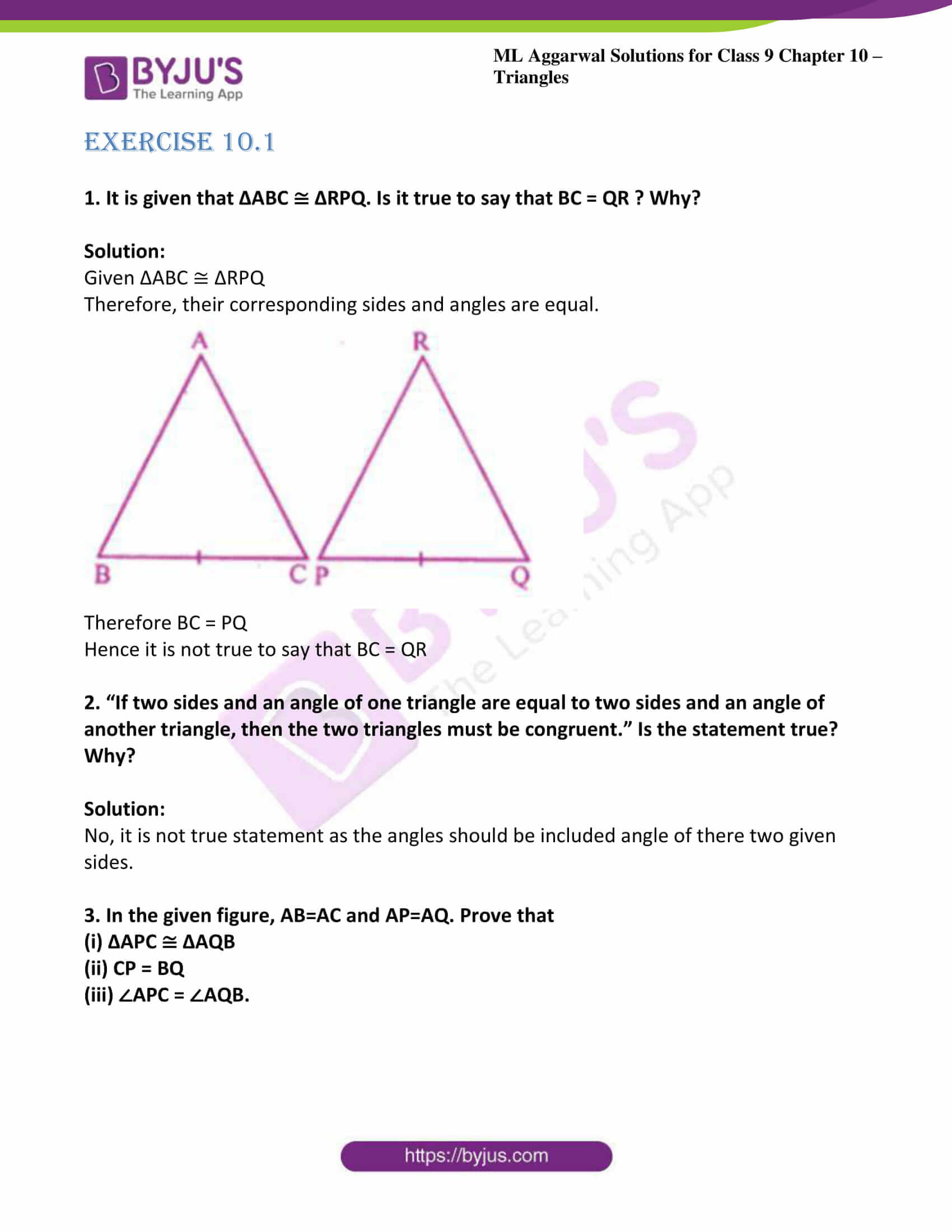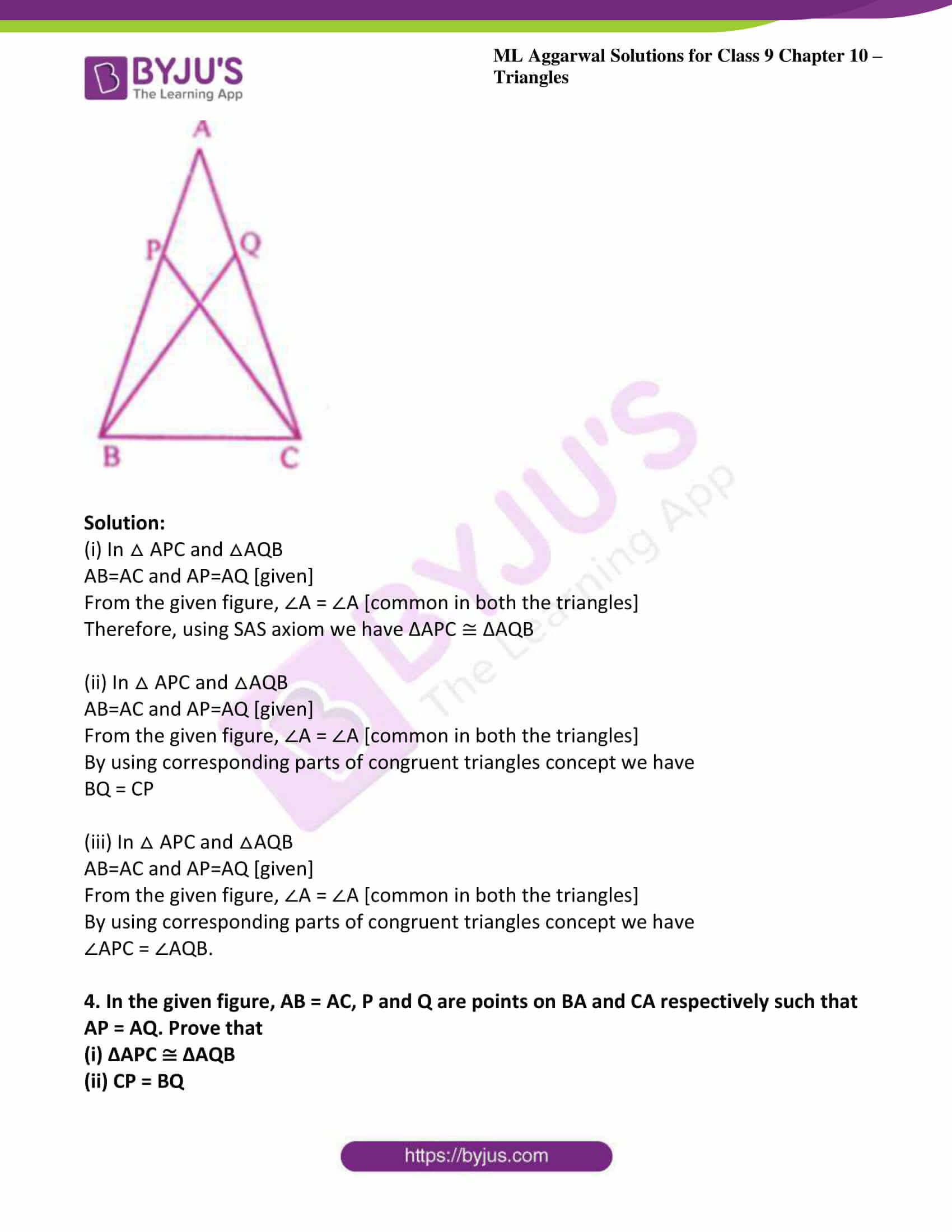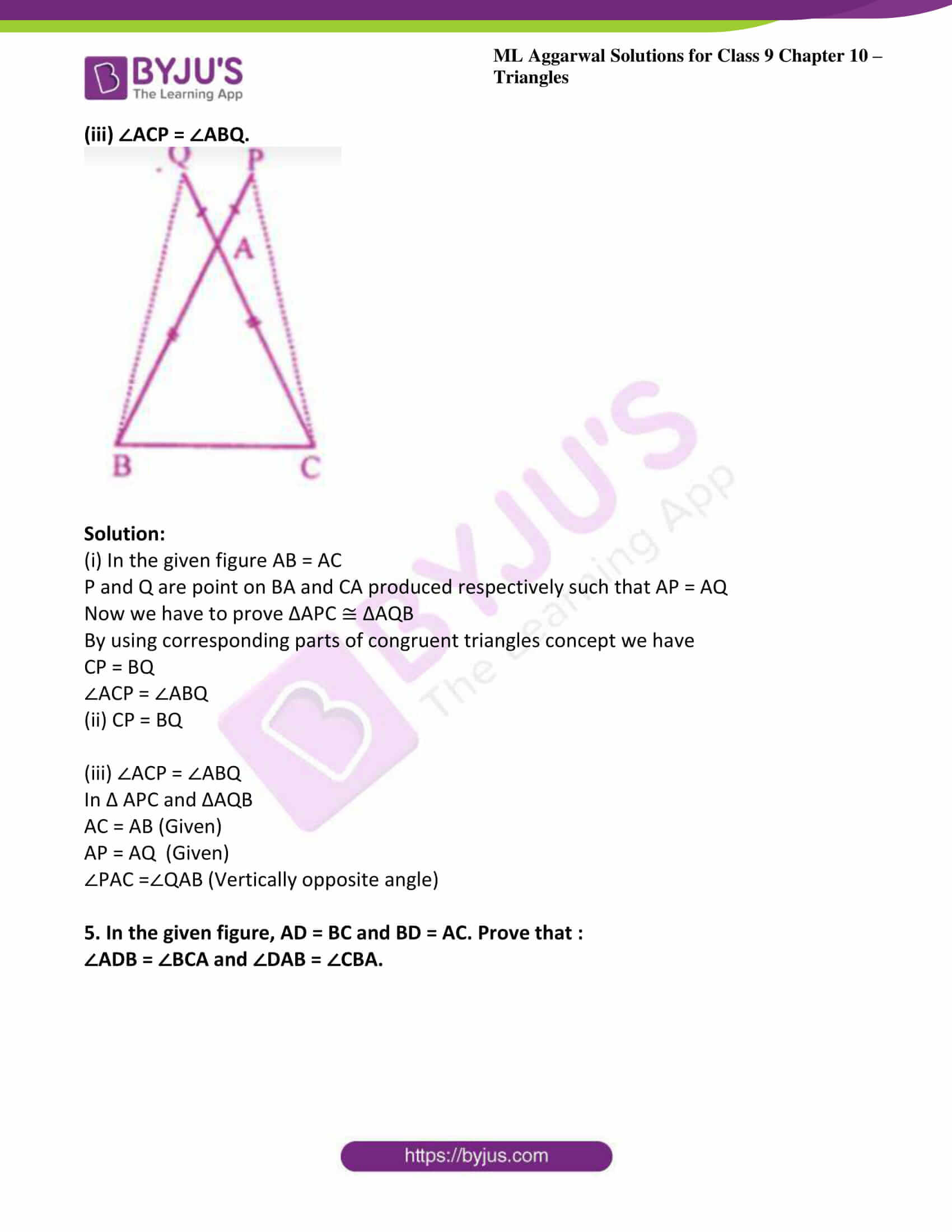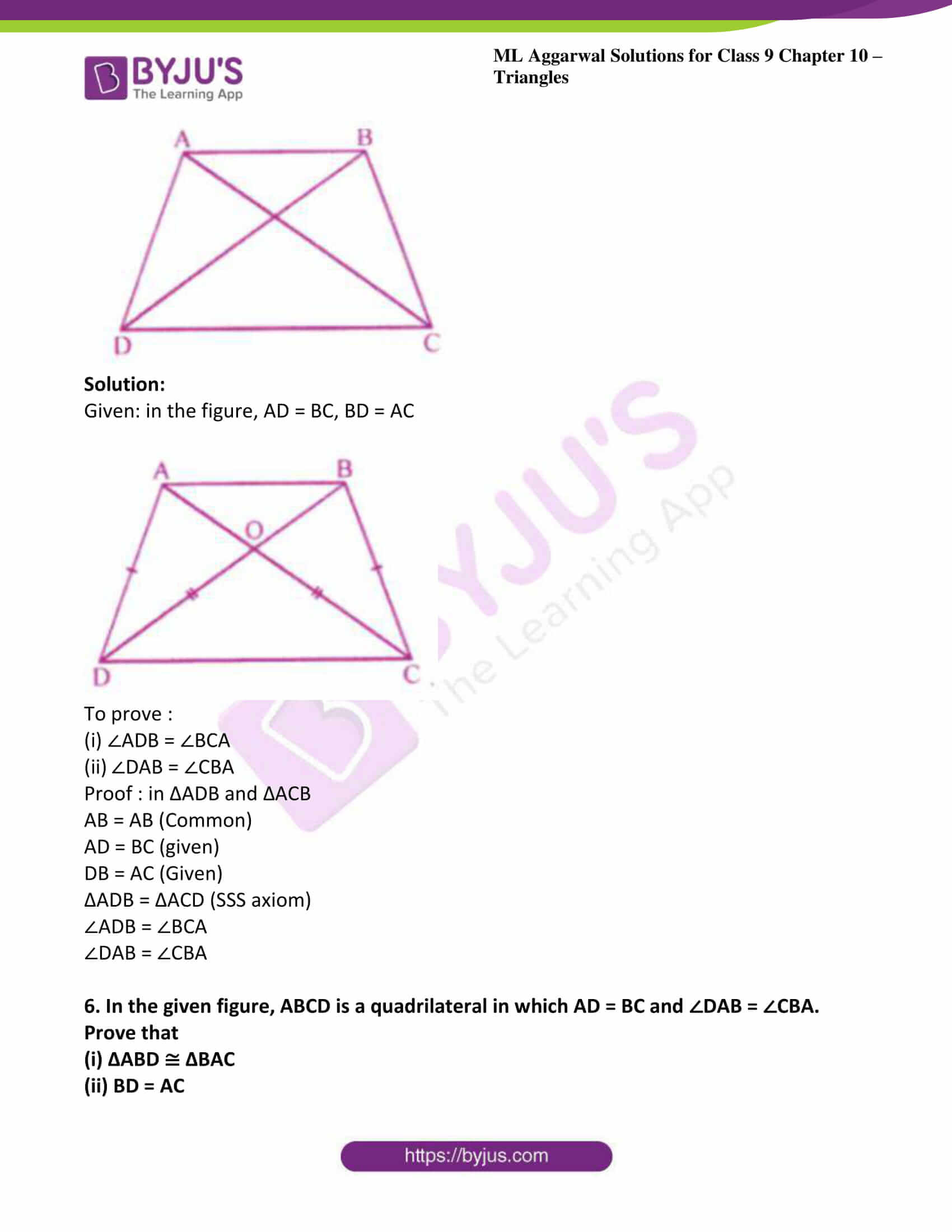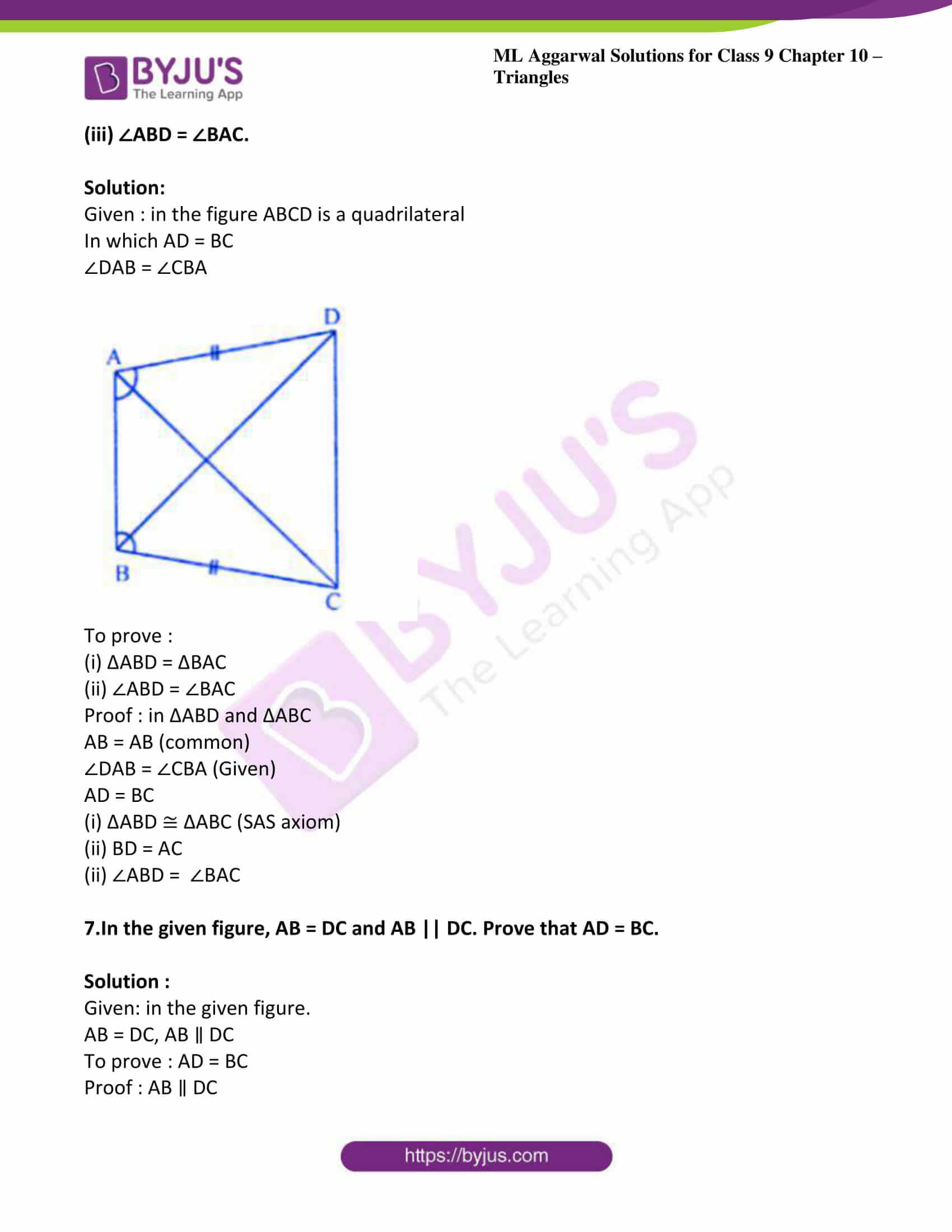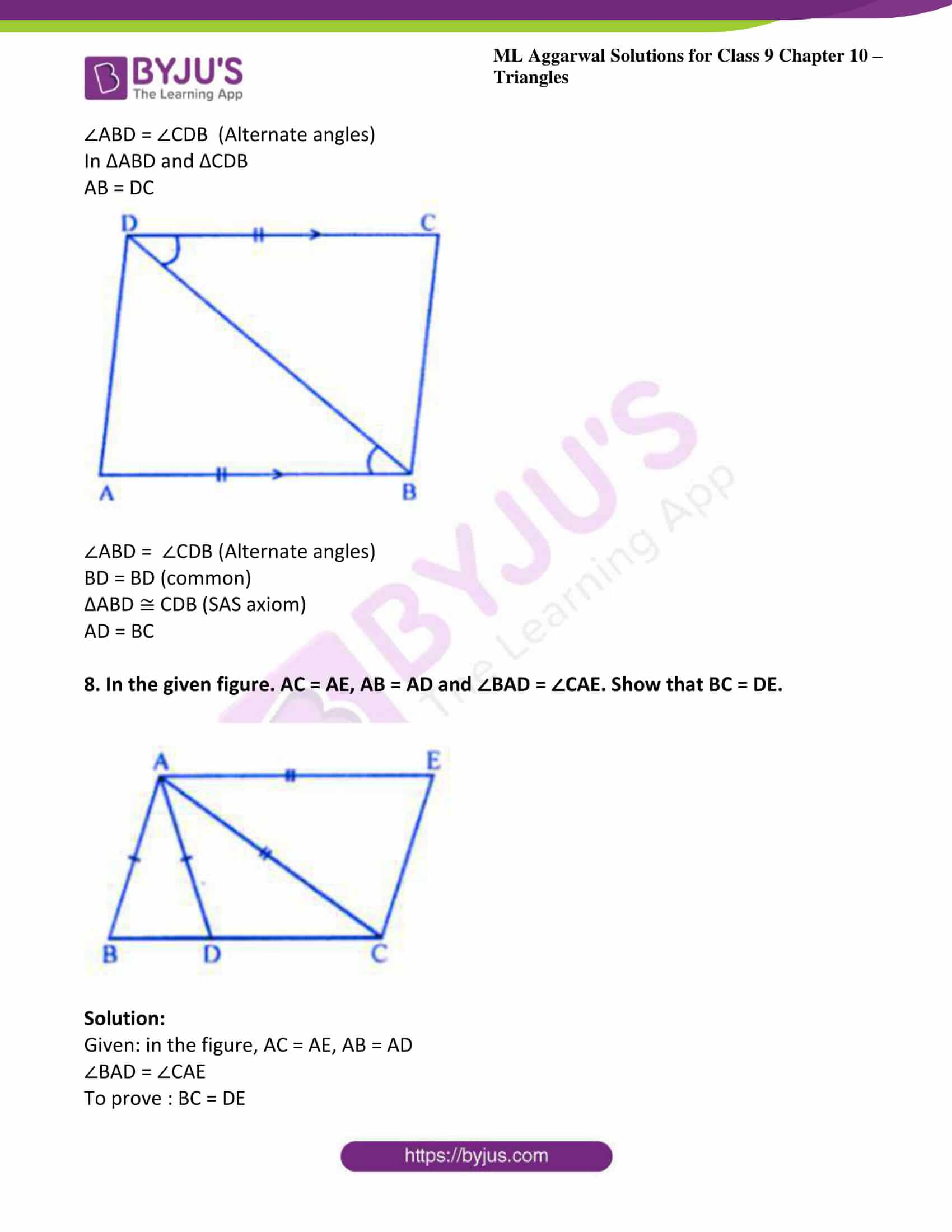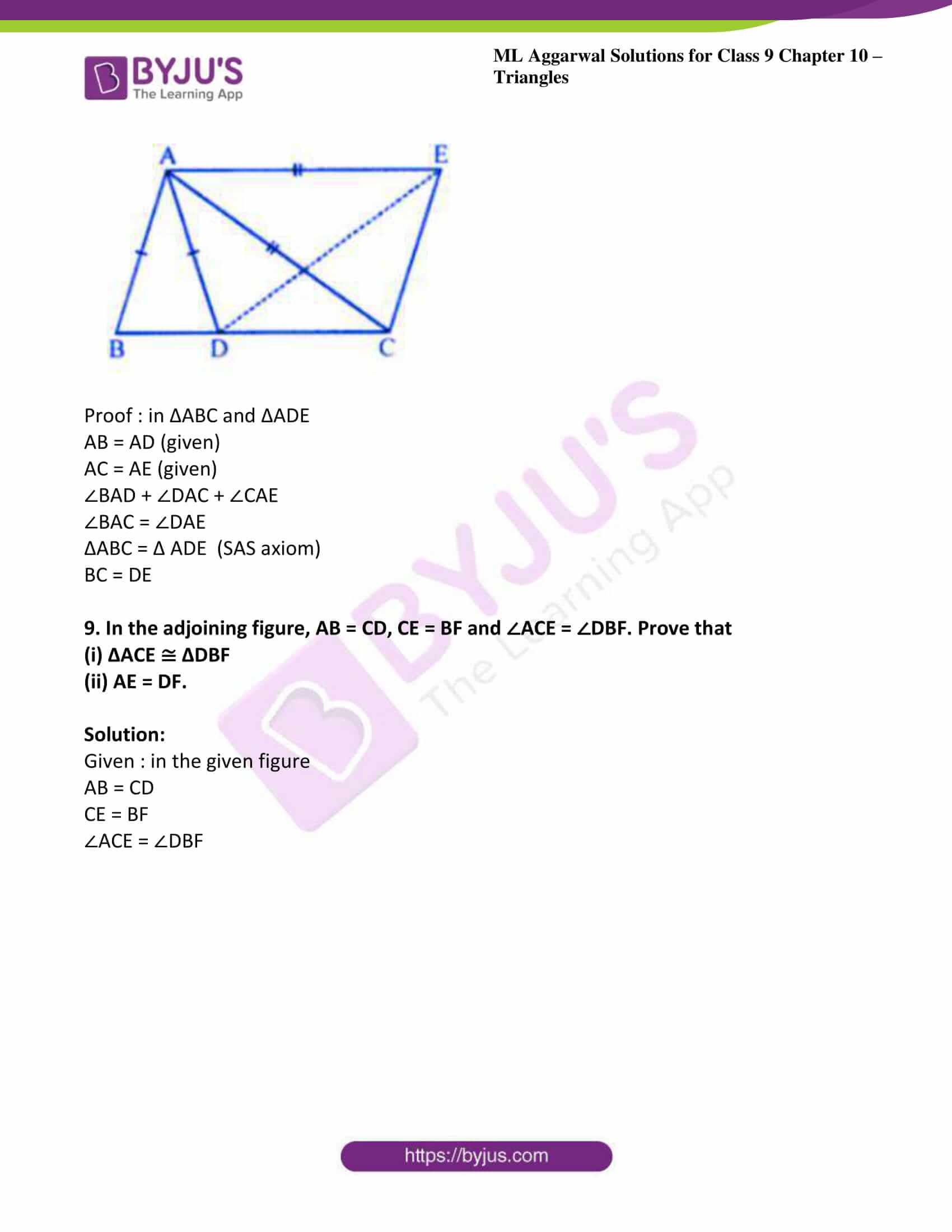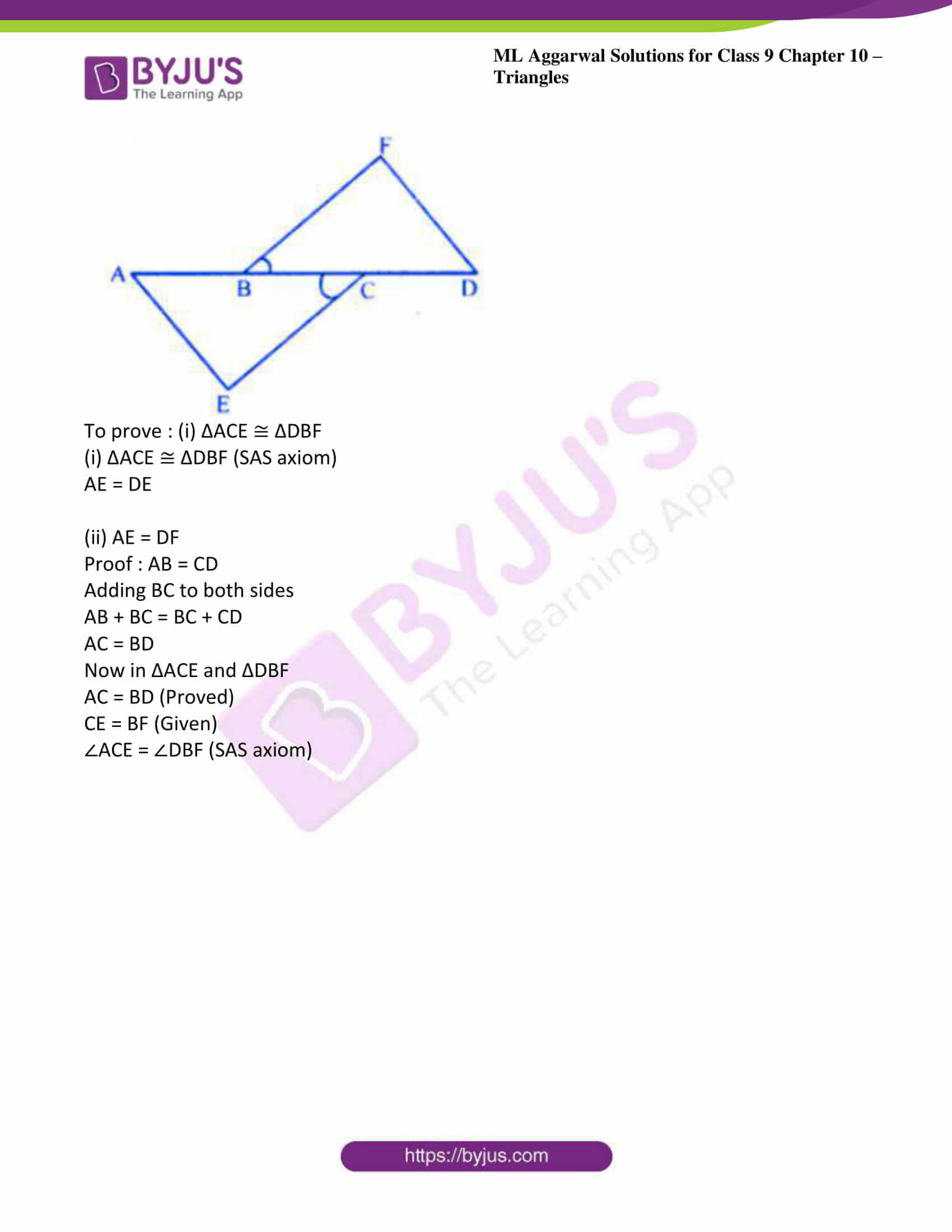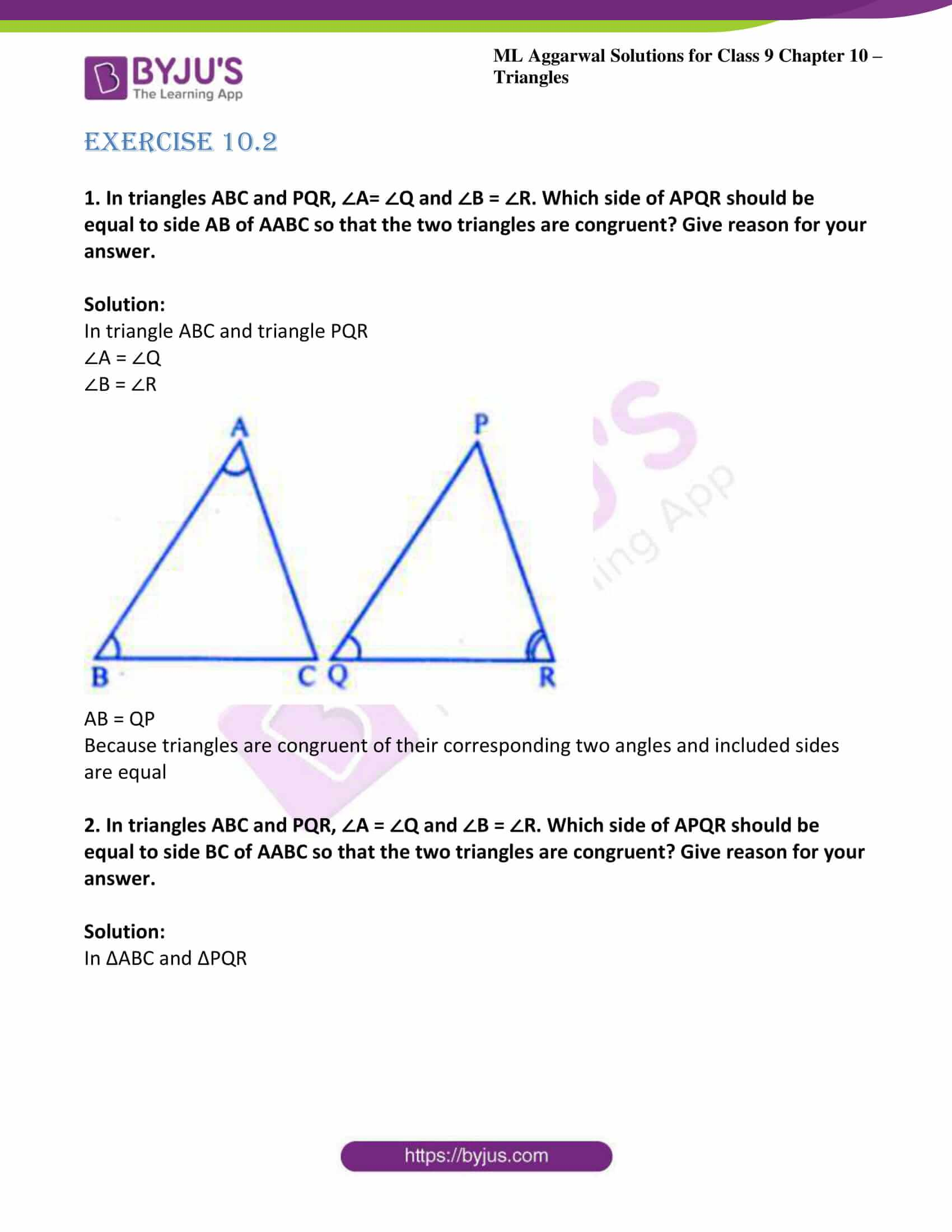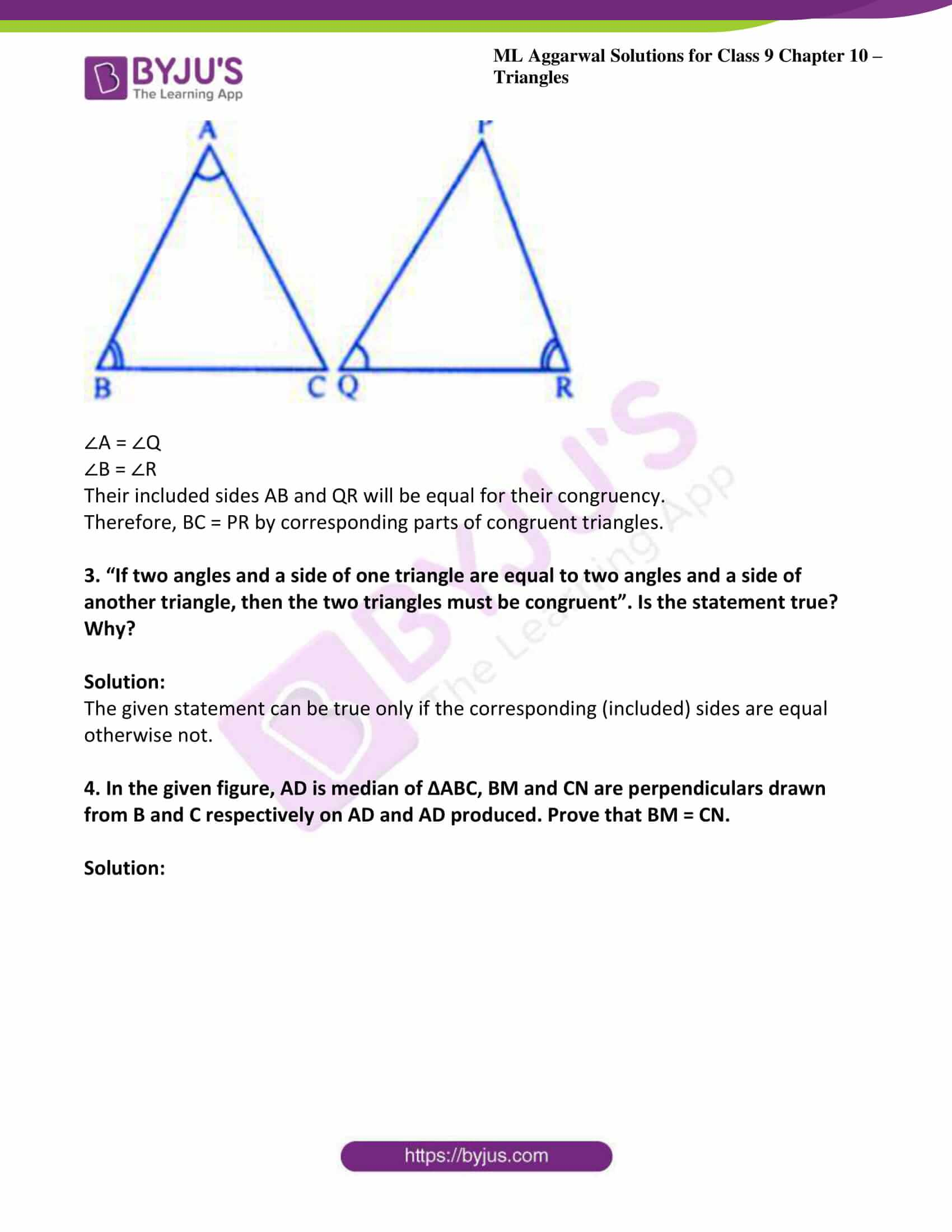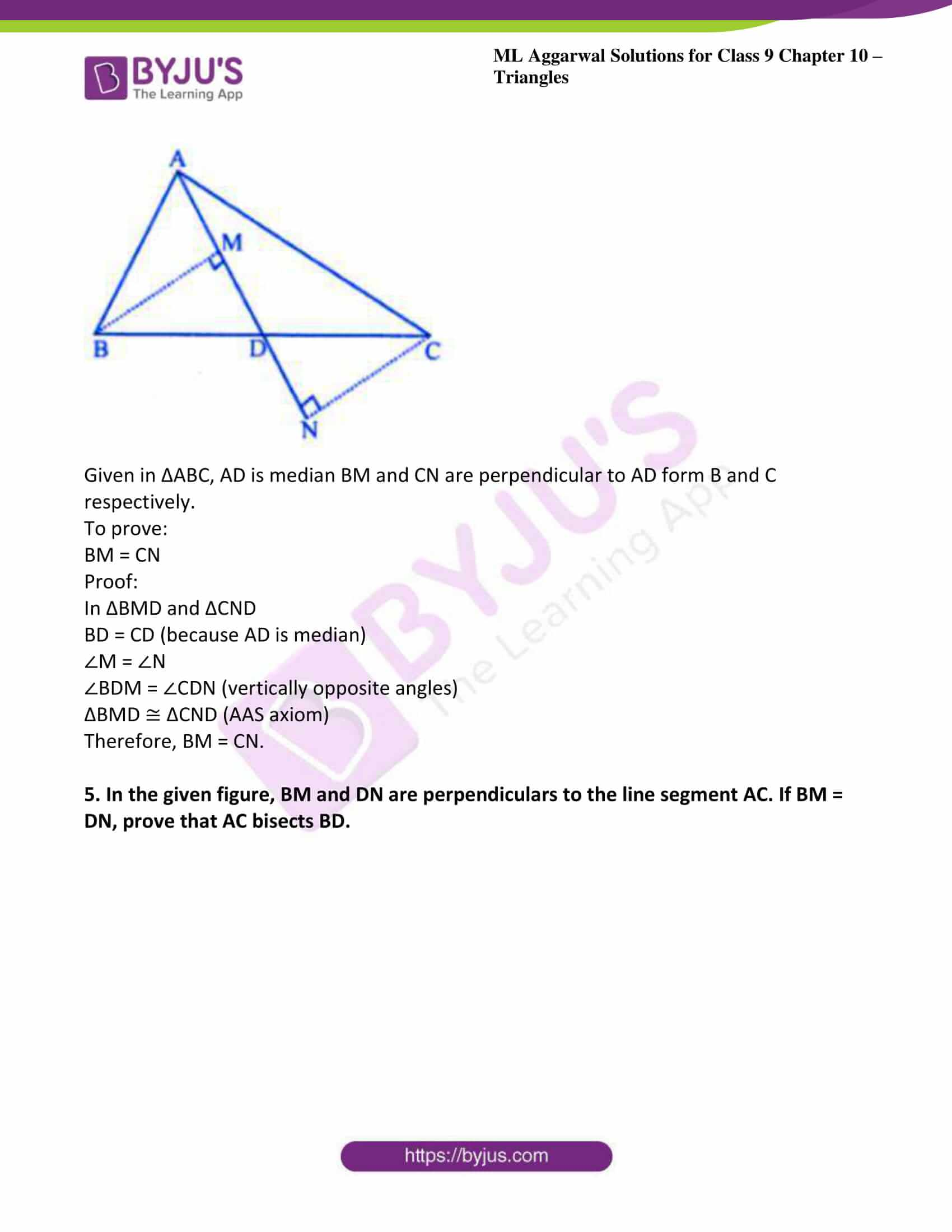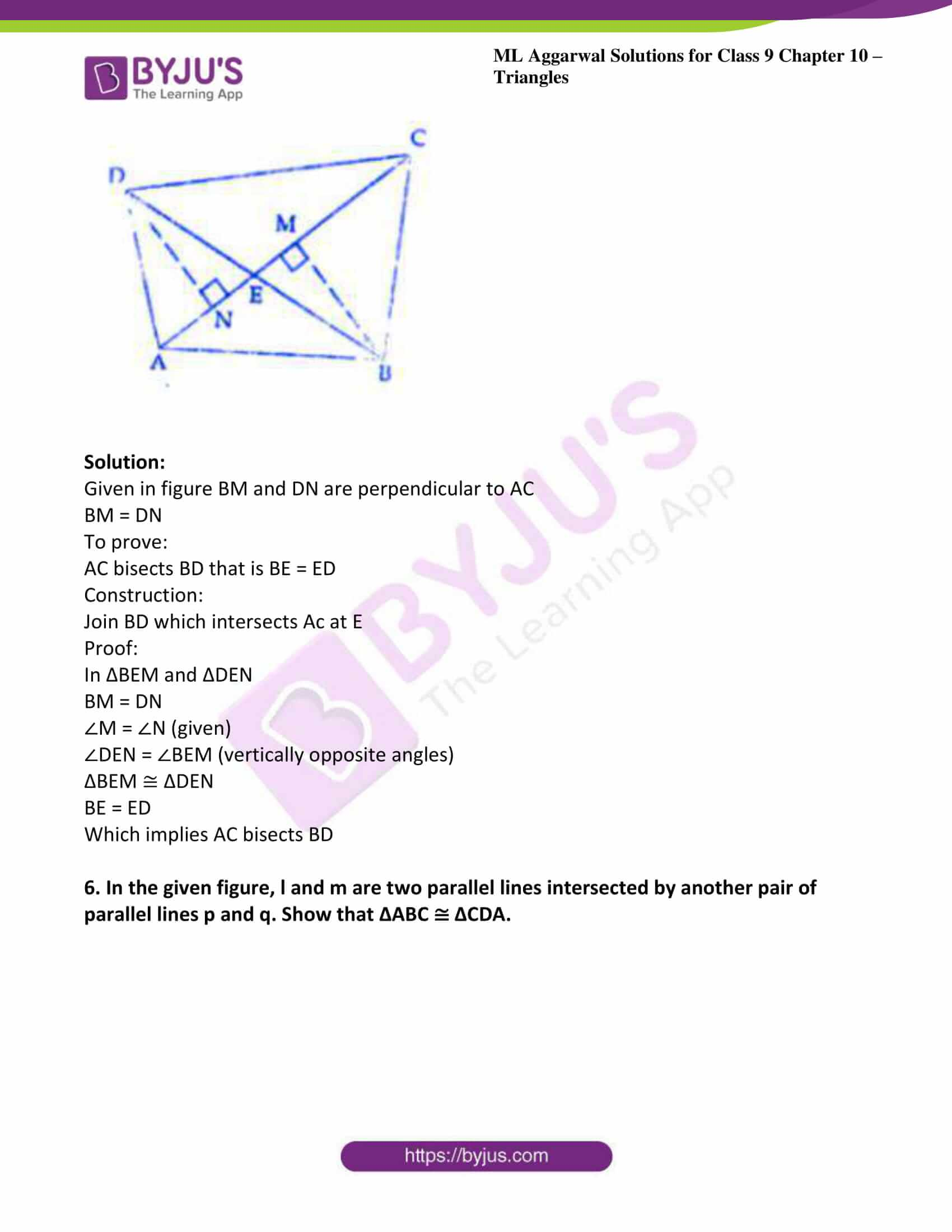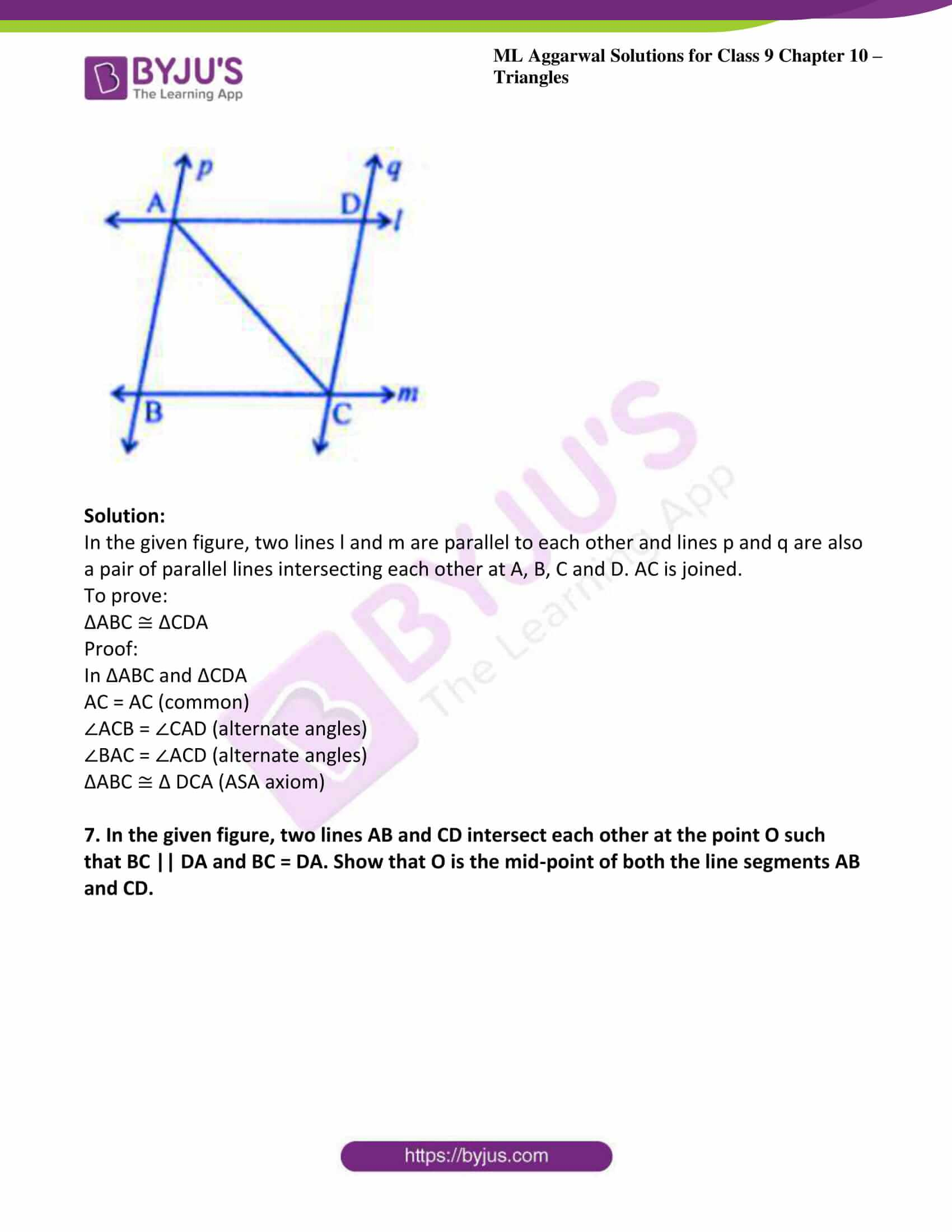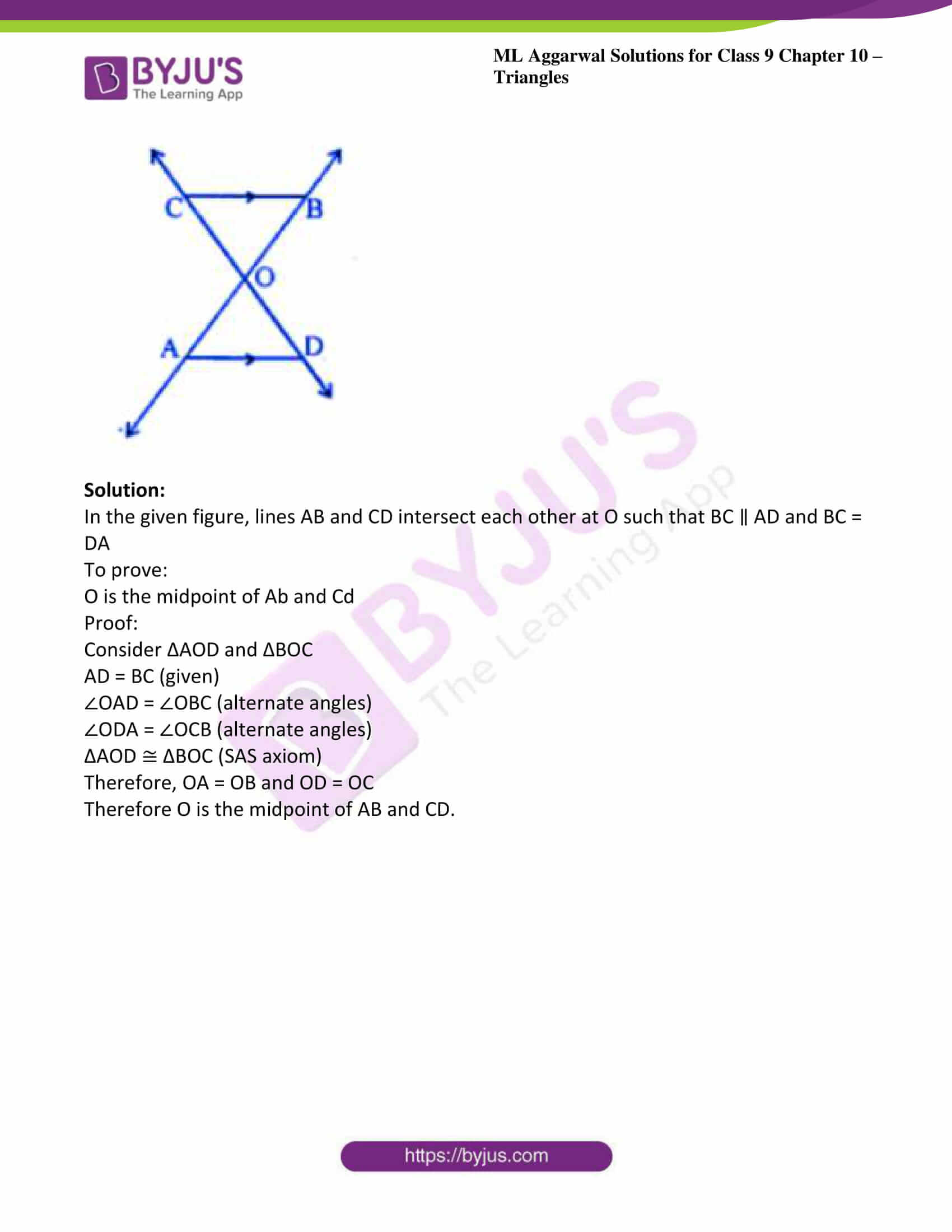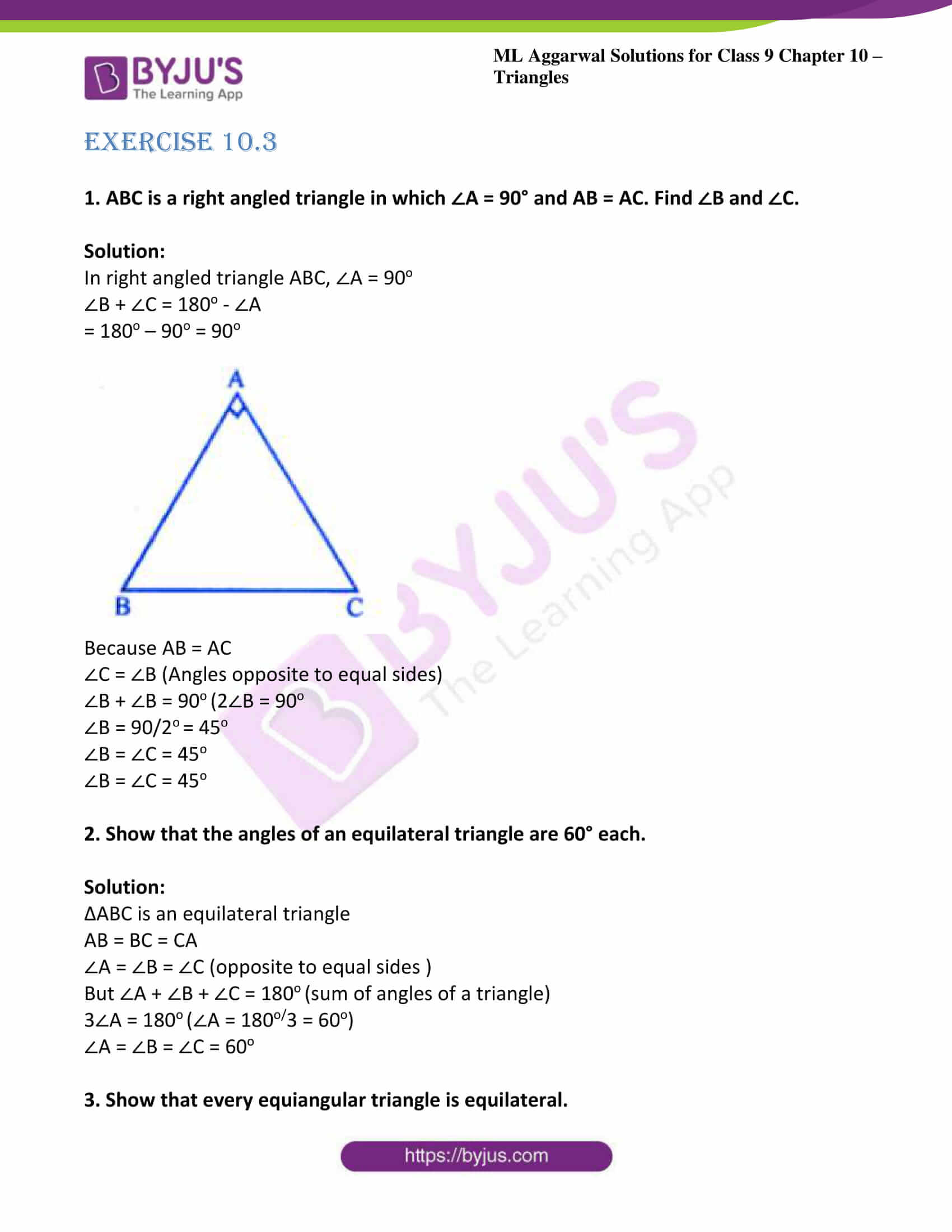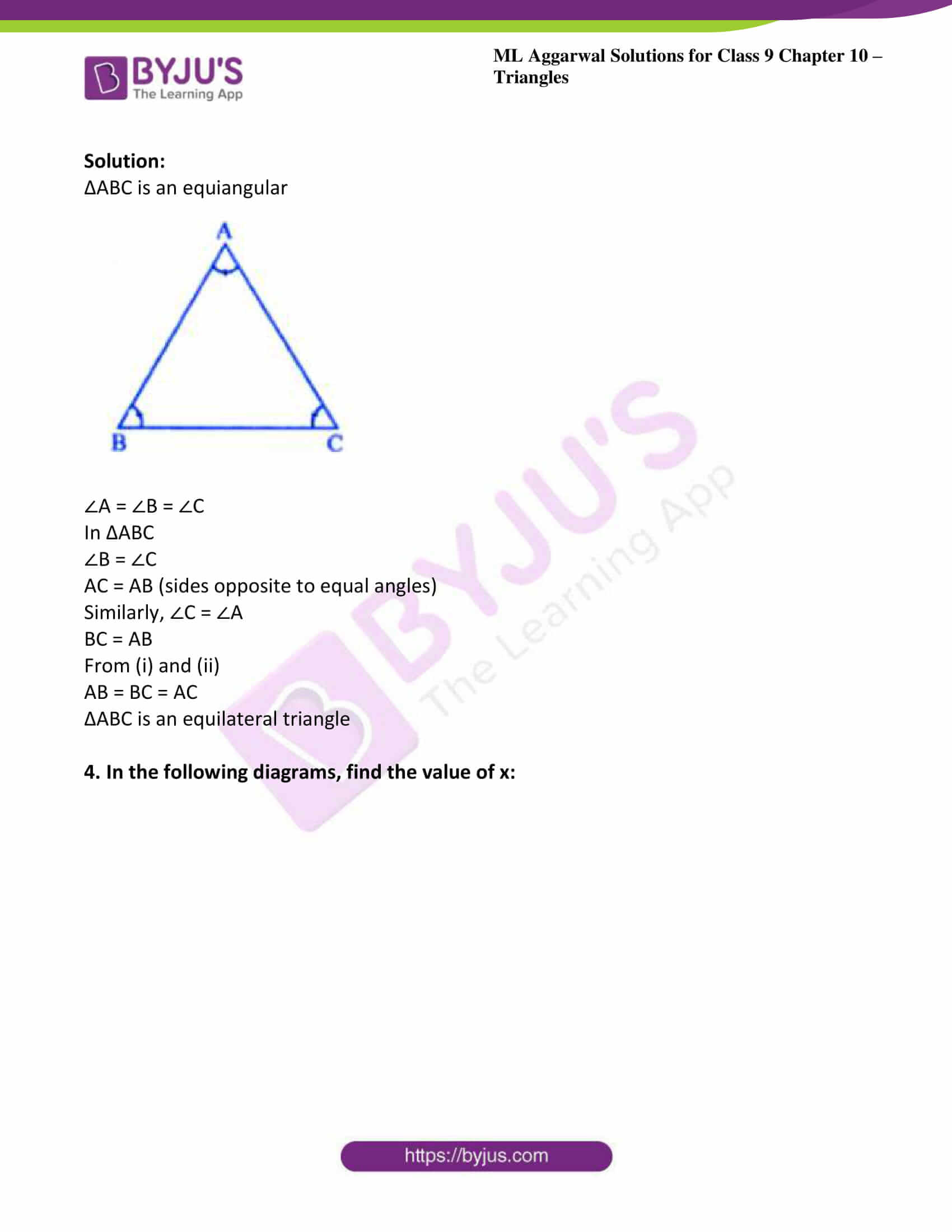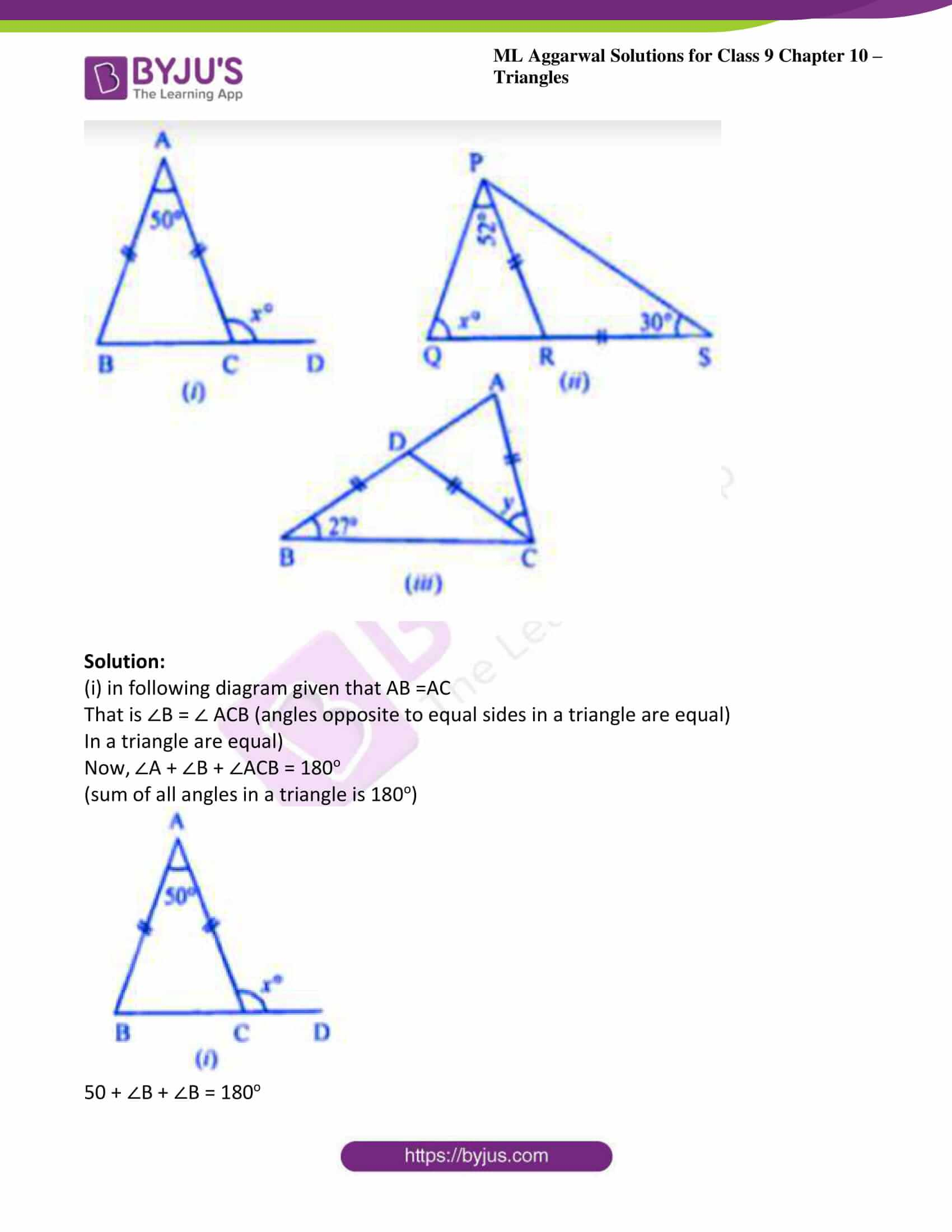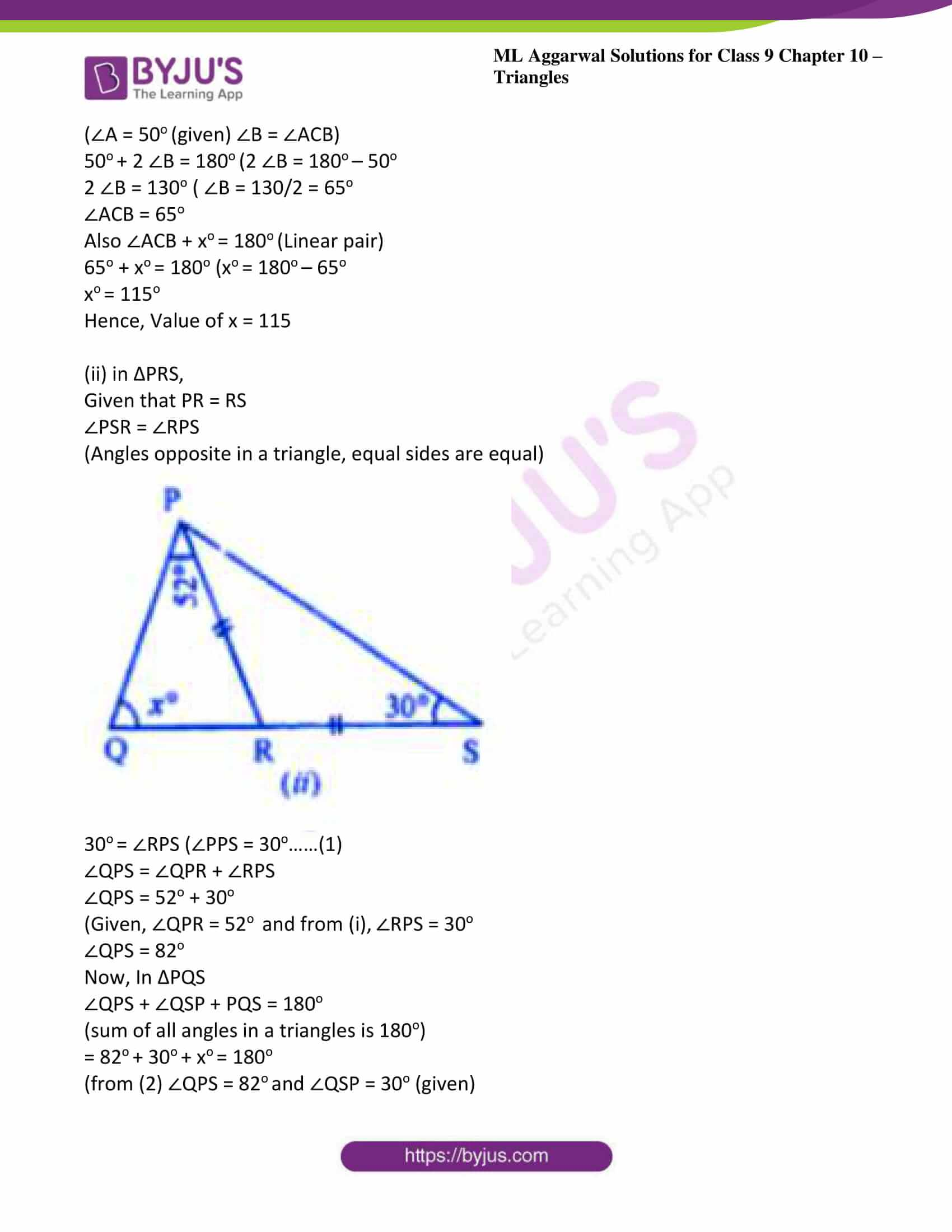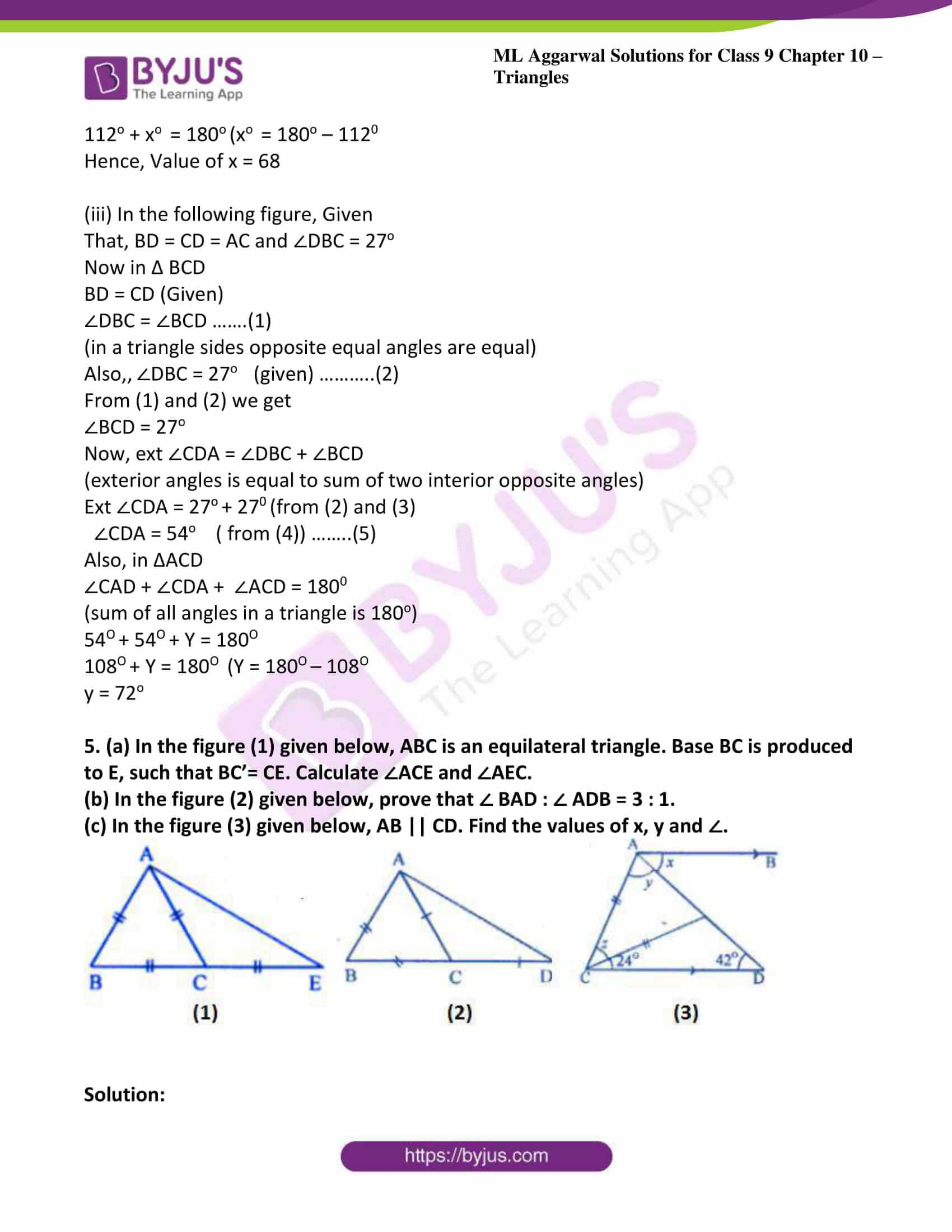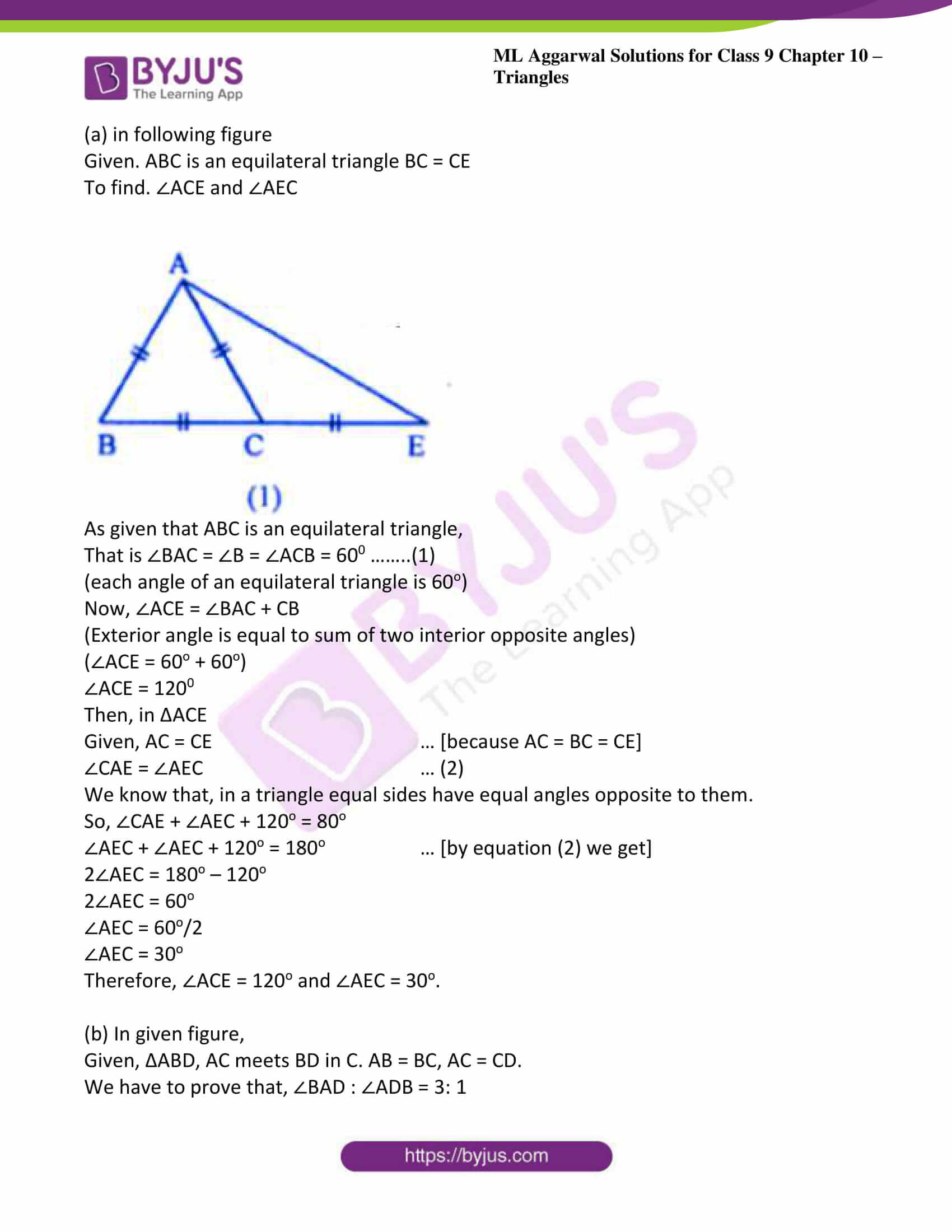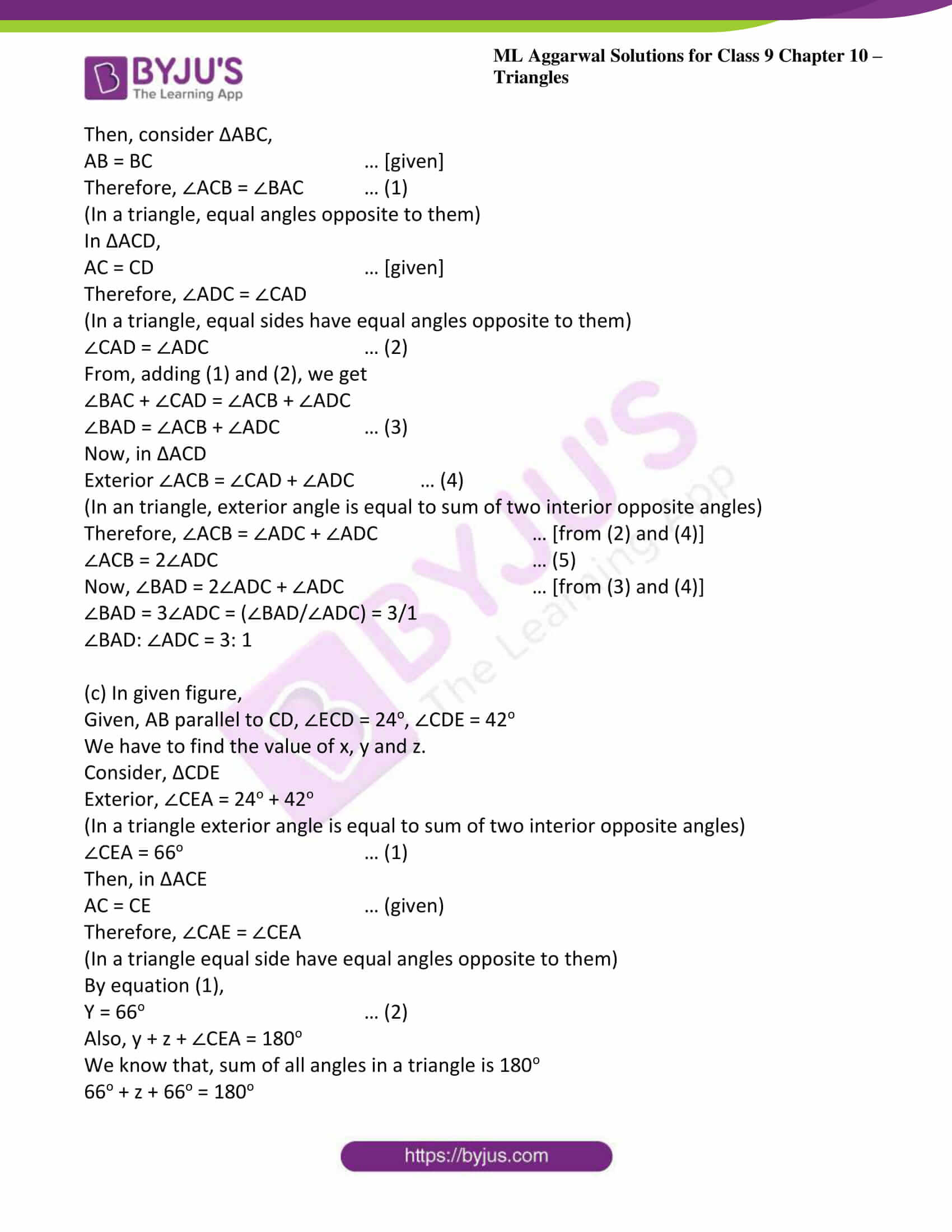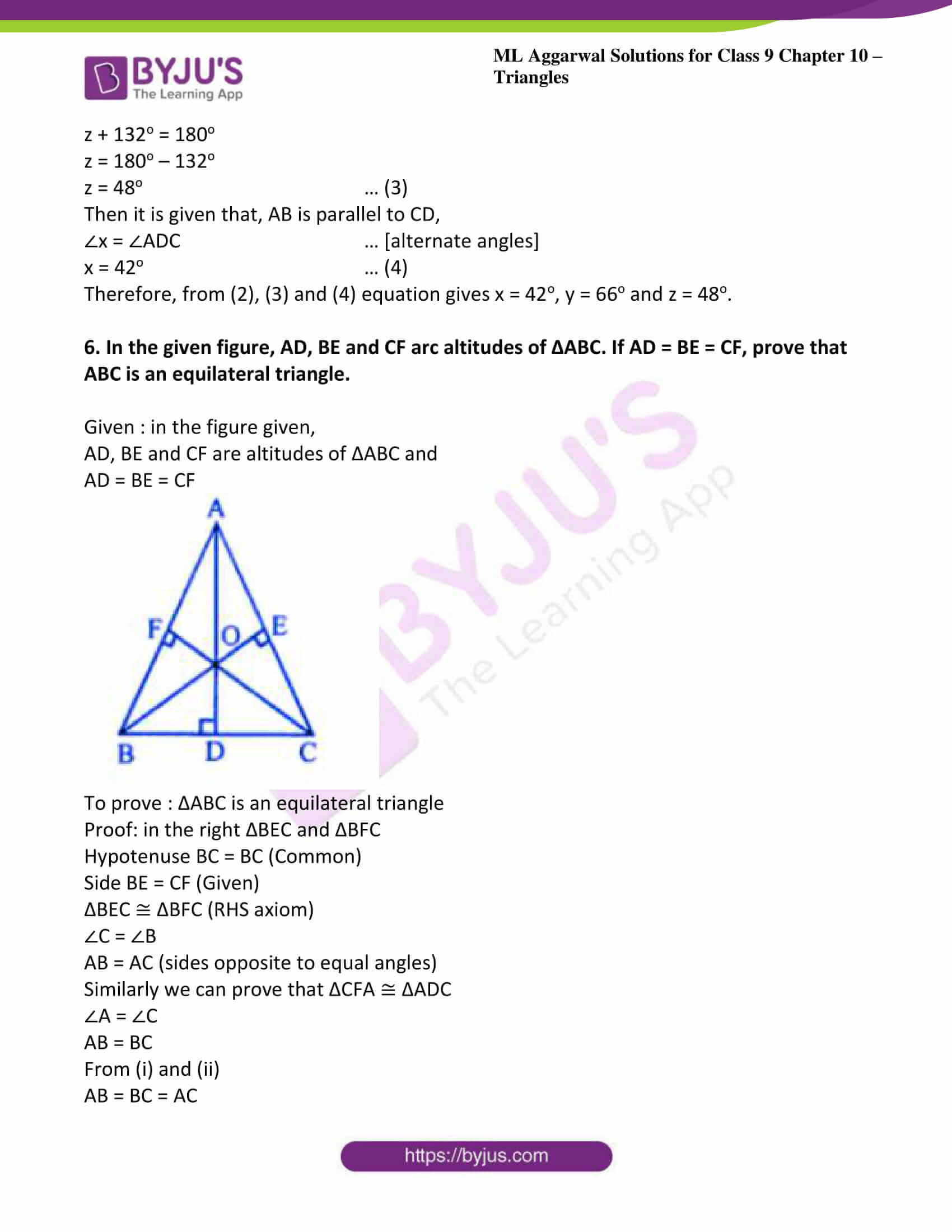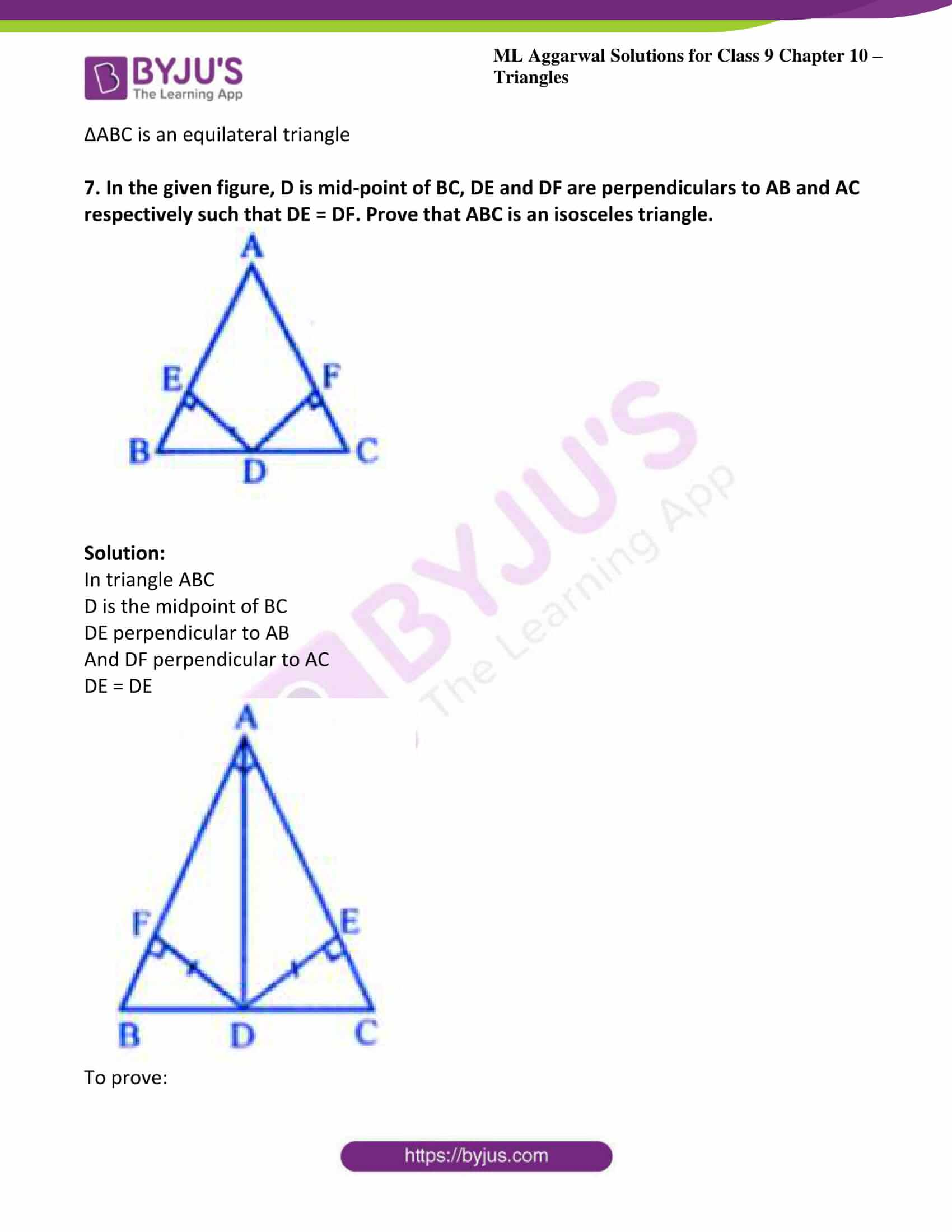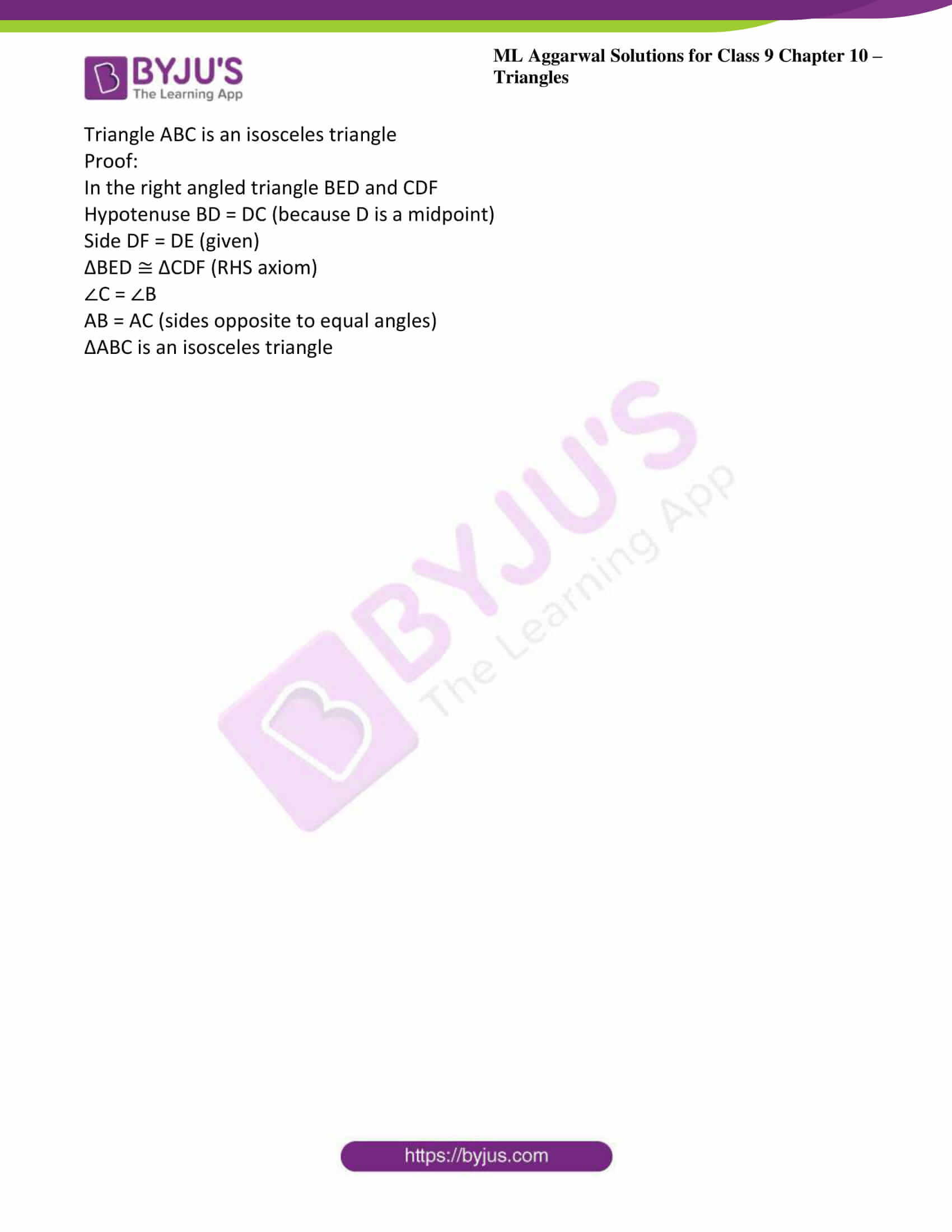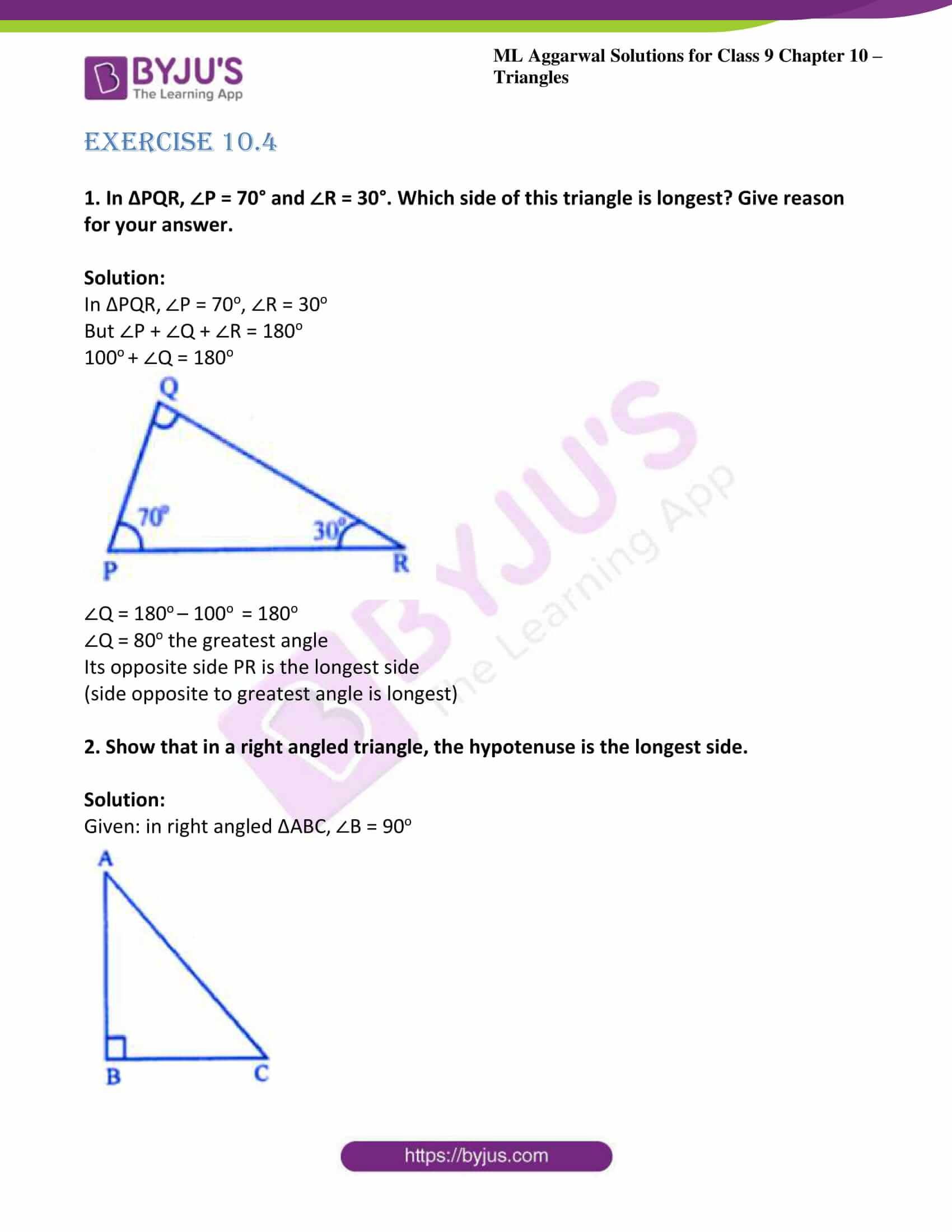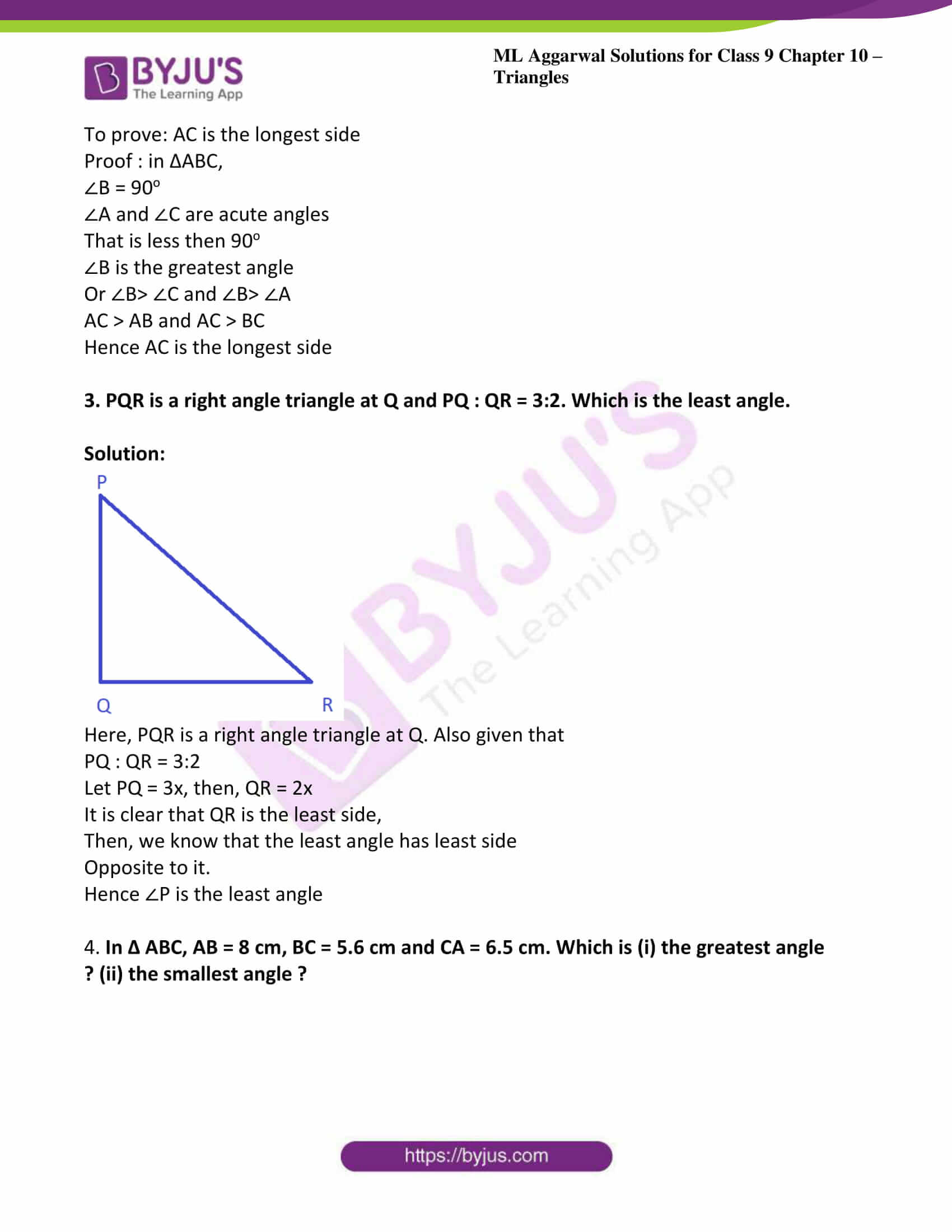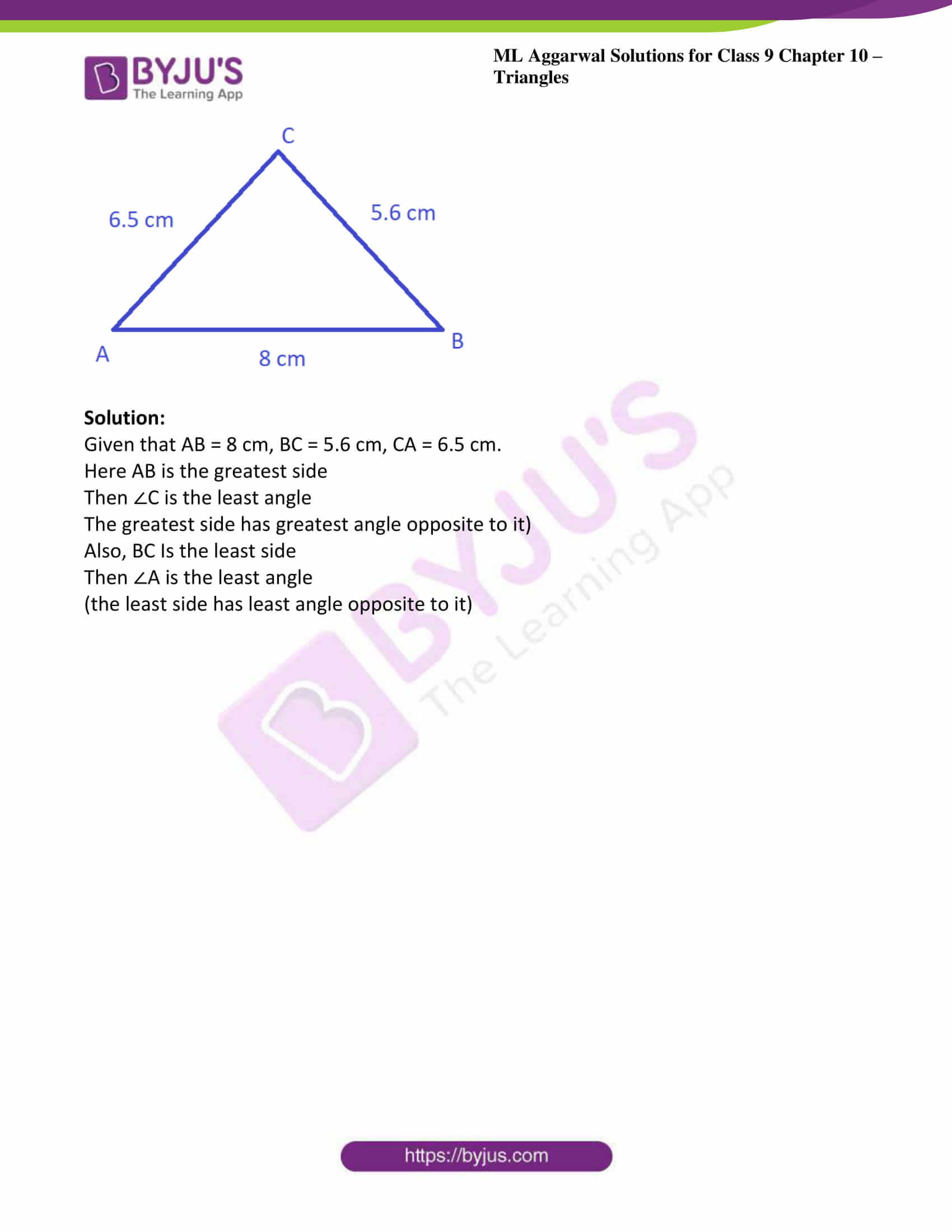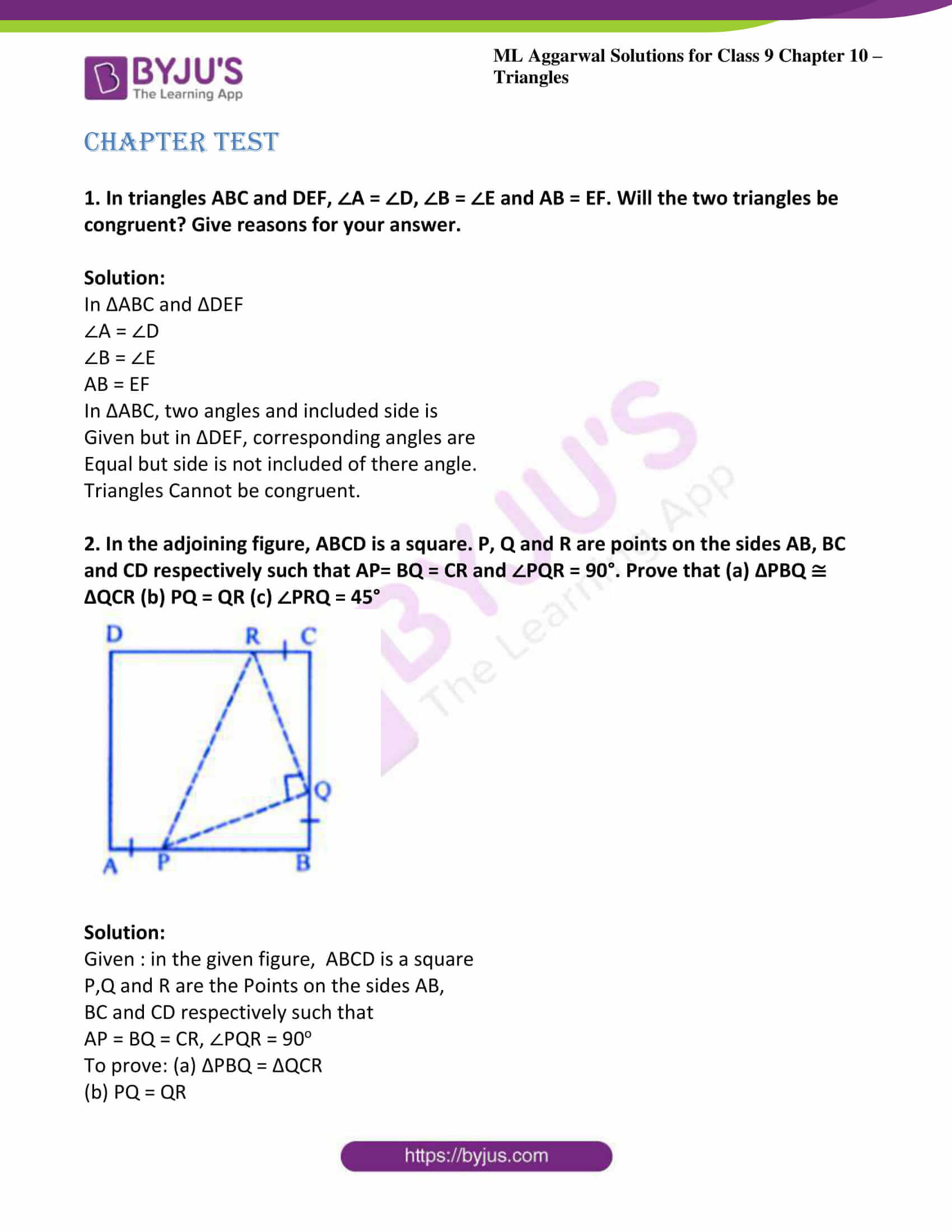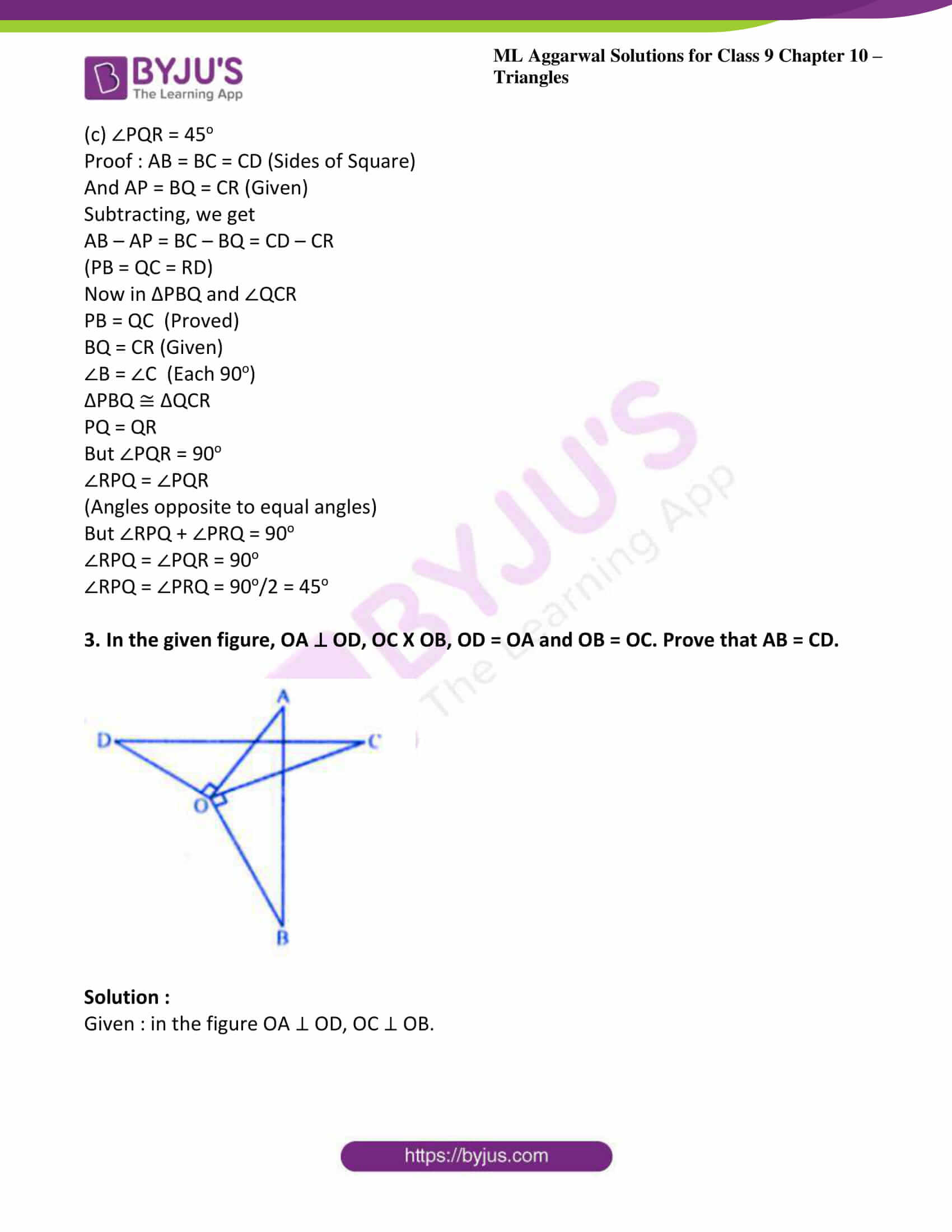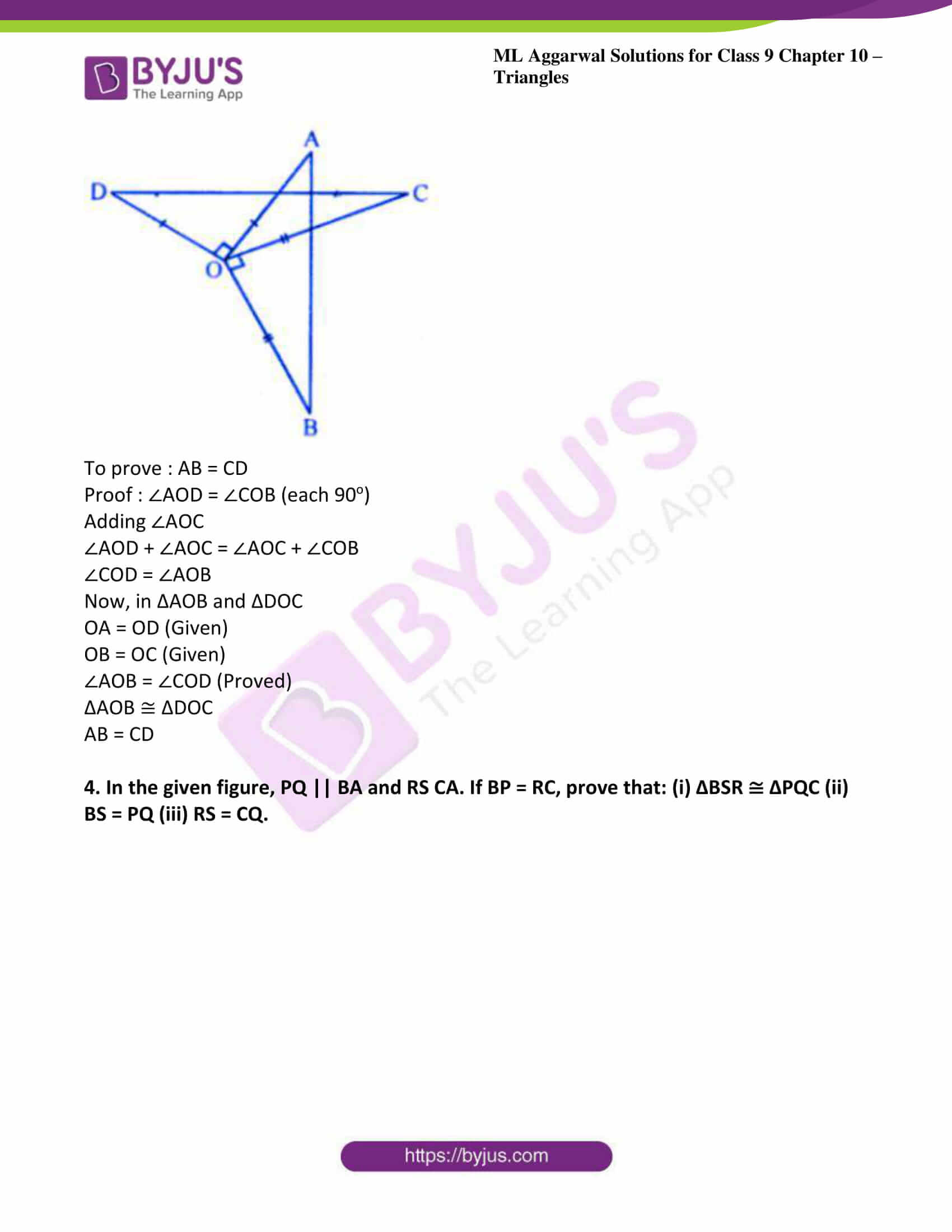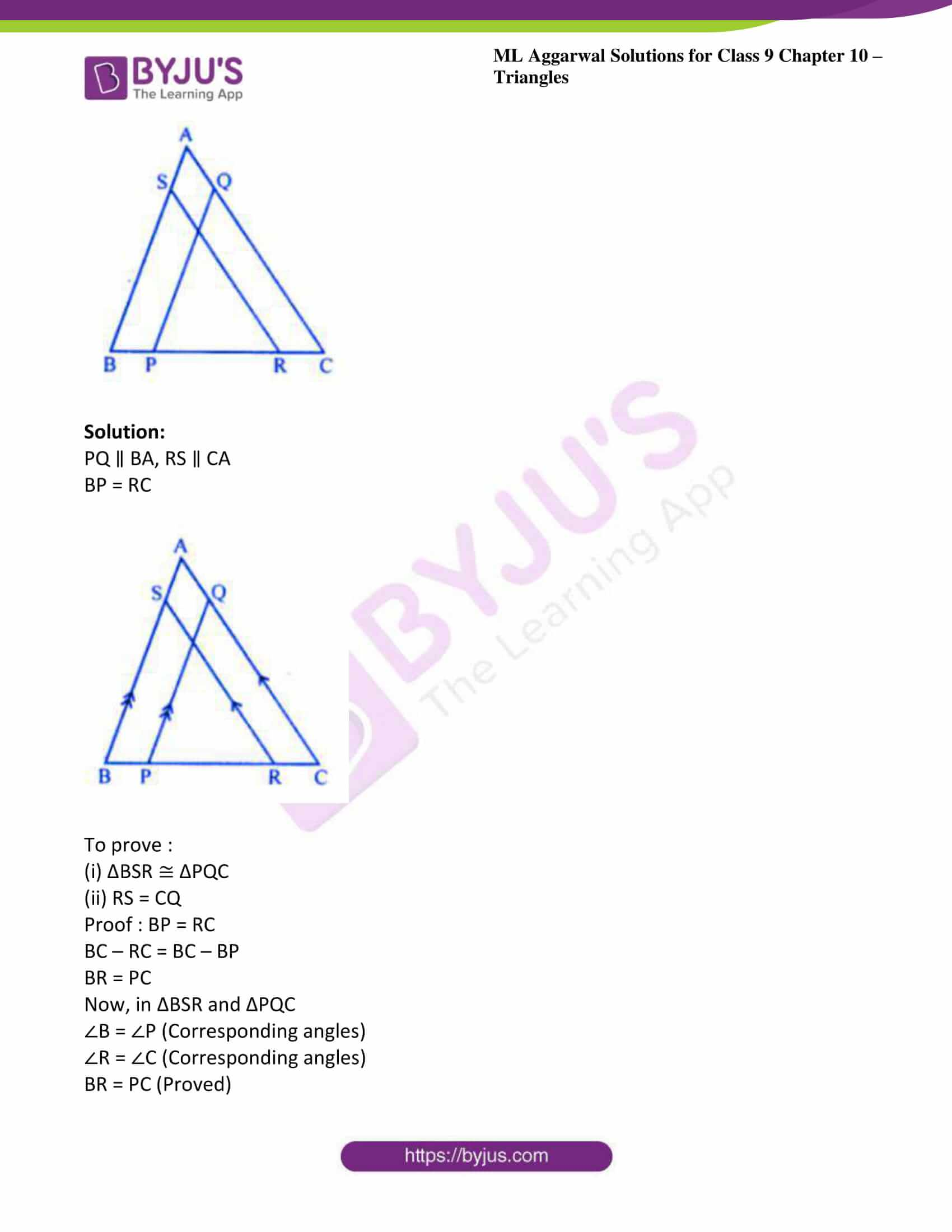### Access answers to ML Aggarwal Solutions for Class 9 Maths Chapter 10 – Triangles

Exercise 10.1

1. It is given that ∆ABC ≅ ∆RPQ. Is it true to say that BC = QR ? Why?

Solution:

Given ∆ABC ≅ ∆RPQ

Therefore, their corresponding sides and angles are equal.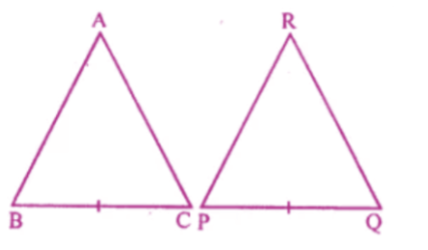Therefore BC = PQ

Hence it is not true to say that BC = QR

2. “If two sides and an angle of one triangle are equal to two sides and an angle of another triangle, then the two triangles must be congruent.” Is the statement true? Why?

Solution:

No, it is not true statement as the angles should be included angle of there two given sides.

3. In the given figure, AB=AC and AP=AQ. Prove that
(i) ∆APC ≅ ∆AQB

(ii) CP = BQ
(iii) ∠APC = ∠AQB.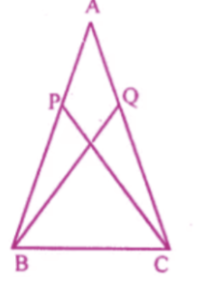Solution:

(i) In △ APC and △AQB

AB=AC and AP=AQ [given]

From the given figure, ∠A = ∠A [common in both the triangles]

Therefore, using SAS axiom we have ∆APC ≅ ∆AQB

(ii) In △ APC and △AQB

AB=AC and AP=AQ [given]

From the given figure, ∠A = ∠A [common in both the triangles]

By using corresponding parts of congruent triangles concept we have

BQ = CP

(iii) In △ APC and △AQB

AB=AC and AP=AQ [given]

From the given figure, ∠A = ∠A [common in both the triangles]

By using corresponding parts of congruent triangles concept we have

∠APC = ∠AQB.

4. In the given figure, AB = AC, P and Q are points on BA and CA respectively such that AP = AQ. Prove that
(i) ∆APC ≅ ∆AQB
(ii) CP = BQ
(iii) ∠ACP = ∠ABQ.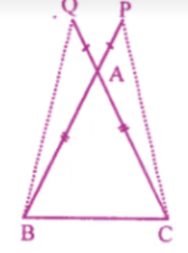Solution:

(i) In the given figure AB = AC

P and Q are point on BA and CA produced respectively such that AP = AQ

Now we have to prove ∆APC ≅ ∆AQB

By using corresponding parts of congruent triangles concept we have

CP = BQ

∠ACP = ∠ABQ

(ii) CP = BQ

(iii) ∠ACP = ∠ABQ

In ∆ APC and ∆AQB

AC = AB (Given)

AP = AQ (Given)

∠PAC =∠QAB (Vertically opposite angle)

5. In the given figure, AD = BC and BD = AC. Prove that :
∠ADB = ∠BCA and ∠DAB = ∠CBA.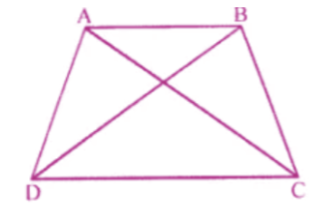Solution:

Given: in the figure, AD = BC, BD = AC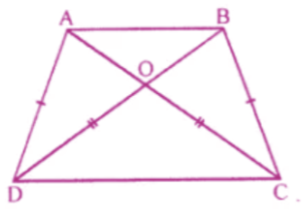To prove :

(ii) ∠DAB = ∠CBA

Proof : in ∆ADB and ∆ACB

AB = AB (Common)

DB = AC (Given)

∠DAB = ∠CBA

6. In the given figure, ABCD is a quadrilateral in which AD = BC and ∠DAB = ∠CBA. Prove that
(i) ∆ABD ≅ ∆BAC
(ii) BD = AC
(iii) ∠ABD = ∠BAC.

Solution:

Given : in the figure ABCD is a quadrilateral

∠DAB = ∠CBA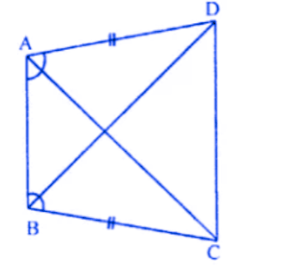To prove :

(i) ∆ABD = ∆BAC

(ii) ∠ABD = ∠BAC

Proof : in ∆ABD and ∆ABC

AB = AB (common)

∠DAB = ∠CBA (Given)

(i) ∆ABD ≅ ∆ABC (SAS axiom)

(ii) BD = AC

(ii) ∠ABD = ∠BAC

7.In the given figure, AB = DC and AB || DC. Prove that AD = BC.

Solution :

Given: in the given figure.

AB = DC, AB ∥ DC

To prove : AD = BC

Proof : AB ∥ DC

∠ABD = ∠CDB (Alternate angles)

In ∆ABD and ∆CDB

AB = DC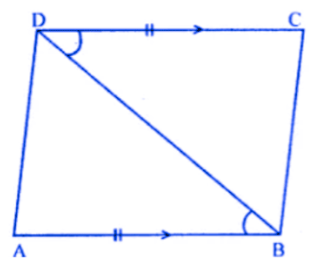∠ABD = ∠CDB (Alternate angles)

BD = BD (common)

∆ABD ≅ CDB (SAS axiom)

8. In the given figure. AC = AE, AB = AD and ∠BAD = ∠CAE. Show that BC = DE.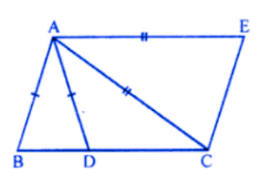Solution:

Given: in the figure, AC = AE, AB = AD

To prove : BC = DE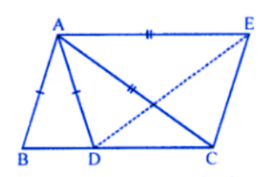Proof : in ∆ABC and ∆ADE

AC = AE (given)

∠BAC = ∠DAE

∆ABC = ∆ ADE (SAS axiom)

BC = DE

9. In the adjoining figure, AB = CD, CE = BF and ∠ACE = ∠DBF. Prove that
(i) ∆ACE ≅ ∆DBF
(ii) AE = DF.

Solution:

Given : in the given figure

AB = CD

CE = BF

∠ACE = ∠DBF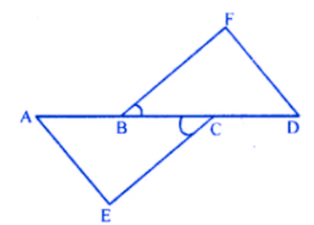To prove : (i) ∆ACE ≅ ∆DBF

(i) ∆ACE ≅ ∆DBF (SAS axiom)

AE = DE

(ii) AE = DF

Proof : AB = CD

AB + BC = BC + CD

AC = BD

Now in ∆ACE and ∆DBF

AC = BD (Proved)

CE = BF (Given)

∠ACE = ∠DBF (SAS axiom)

Exercise 10.2

1. In triangles ABC and PQR, ∠A= ∠Q and ∠B = ∠R. Which side of APQR should be equal to side AB of AABC so that the two triangles are congruent? Give reason for your answer.

Solution:

In triangle ABC and triangle PQR

∠A = ∠Q

∠B = ∠R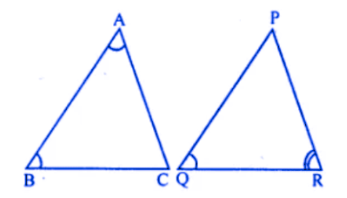AB = QP

Because triangles are congruent of their corresponding two angles and included sides are equal

2. In triangles ABC and PQR, ∠A = ∠Q and ∠B = ∠R. Which side of APQR should be equal to side BC of AABC so that the two triangles are congruent? Give reason for your answer.

Solution:

In ∆ABC and ∆PQR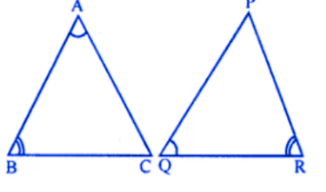∠A = ∠Q

∠B = ∠R

Their included sides AB and QR will be equal for their congruency.

Therefore, BC = PR by corresponding parts of congruent triangles.

3. “If two angles and a side of one triangle are equal to two angles and a side of another triangle, then the two triangles must be congruent”. Is the statement true? Why?

Solution:

The given statement can be true only if the corresponding (included) sides are equal otherwise not.

4. In the given figure, AD is median of ∆ABC, BM and CN are perpendiculars drawn from B and C respectively on AD and AD produced. Prove that BM = CN.

Solution: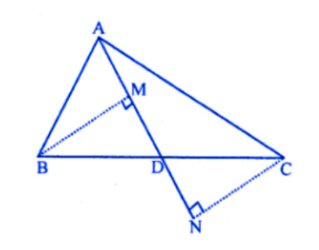Given in ∆ABC, AD is median BM and CN are perpendicular to AD form B and C respectively.

To prove:

BM = CN

Proof:

In ∆BMD and ∆CND

BD = CD (because AD is median)

∠M = ∠N

∠BDM = ∠CDN (vertically opposite angles)

∆BMD ≅ ∆CND (AAS axiom)

Therefore, BM = CN.

5. In the given figure, BM and DN are perpendiculars to the line segment AC. If BM = DN, prove that AC bisects BD.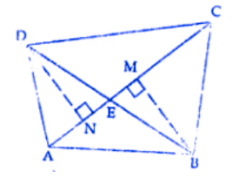Solution:

Given in figure BM and DN are perpendicular to AC

BM = DN

To prove:

AC bisects BD that is BE = ED

Construction:

Join BD which intersects Ac at E

Proof:

In ∆BEM and ∆DEN

BM = DN

∠M = ∠N (given)

∠DEN = ∠BEM (vertically opposite angles)

∆BEM ≅ ∆DEN

BE = ED

Which implies AC bisects BD

6. In the given figure, l and m are two parallel lines intersected by another pair of parallel lines p and q. Show that ∆ABC ≅ ∆CDA.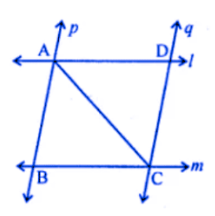Solution:

In the given figure, two lines l and m are parallel to each other and lines p and q are also a pair of parallel lines intersecting each other at A, B, C and D. AC is joined.

To prove:

∆ABC ≅ ∆CDA

Proof:

In ∆ABC and ∆CDA

AC = AC (common)

∠BAC = ∠ACD (alternate angles)

∆ABC ≅ ∆ DCA (ASA axiom)

7. In the given figure, two lines AB and CD intersect each other at the point O such that BC || DA and BC = DA. Show that O is the mid-point of both the line segments AB and CD.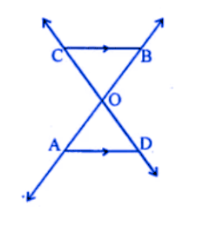Solution:

In the given figure, lines AB and CD intersect each other at O such that BC ∥ AD and BC = DA

To prove:

O is the midpoint of Ab and Cd

Proof:

Consider ∆AOD and ∆BOC

∠ODA = ∠OCB (alternate angles)

∆AOD ≅ ∆BOC (SAS axiom)

Therefore, OA = OB and OD = OC

Therefore O is the midpoint of AB and CD.

Exercise 10.3

1. ABC is a right angled triangle in which ∠A = 90° and AB = AC. Find ∠B and ∠C.

Solution:

In right angled triangle ABC, ∠A = 90o

∠B + ∠C = 180o – ∠A

= 180o – 90o = 90o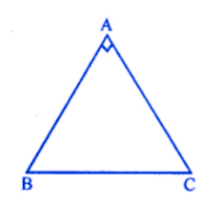Because AB = AC

∠C = ∠B (Angles opposite to equal sides)

∠B + ∠B = 90o (2∠B = 90o

∠B = 90/2o = 45o

∠B = ∠C = 45o

∠B = ∠C = 45o

2. Show that the angles of an equilateral triangle are 60° each.

Solution:

∆ABC is an equilateral triangle

AB = BC = CA

∠A = ∠B = ∠C (opposite to equal sides )

But ∠A + ∠B + ∠C = 180o (sum of angles of a triangle)

3∠A = 180o (∠A = 180o/3 = 60o)

∠A = ∠B = ∠C = 60o

3. Show that every equiangular triangle is equilateral.

Solution:

∆ABC is an equiangular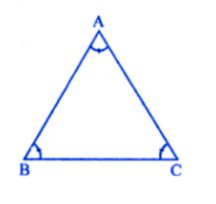∠A = ∠B = ∠C

In ∆ABC

∠B = ∠C

AC = AB (sides opposite to equal angles)

Similarly, ∠C = ∠A

BC = AB

From (i) and (ii)

AB = BC = AC

∆ABC is an equilateral triangle

4. In the following diagrams, find the value of x: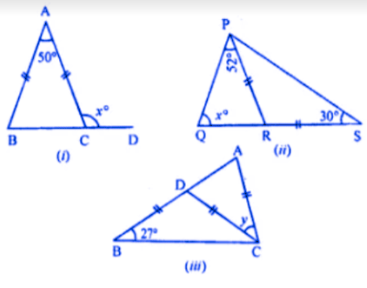Solution:

(i) in following diagram given that AB =AC

That is ∠B = ∠ ACB (angles opposite to equal sides in a triangle are equal)

In a triangle are equal)

Now, ∠A + ∠B + ∠ACB = 180o

(sum of all angles in a triangle is 180o)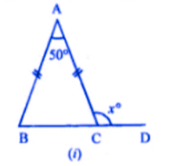50 + ∠B + ∠B = 180o

(∠A = 50o (given) ∠B = ∠ACB)

50o + 2 ∠B = 180o (2 ∠B = 180o – 50o

2 ∠B = 130o ( ∠B = 130/2 = 65o

∠ACB = 65o

Also ∠ACB + xo = 180o (Linear pair)

65o + xo = 180o (xo = 180o – 65o

xo = 115o

Hence, Value of x = 115

(ii) in ∆PRS,

Given that PR = RS

∠PSR = ∠RPS

(Angles opposite in a triangle, equal sides are equal)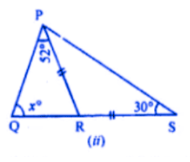30o = ∠RPS (∠PPS = 30o……(1)

∠QPS = ∠QPR + ∠RPS

∠QPS = 52o + 30o

(Given, ∠QPR = 52o and from (i), ∠RPS = 30o

∠QPS = 82o

Now, In ∆PQS

∠QPS + ∠QSP + PQS = 180o

(sum of all angles in a triangles is 180o)

= 82o + 30o + xo = 180o

(from (2) ∠QPS = 82o and ∠QSP = 30o (given)

112o + xo = 180o (xo = 180o – 1120

Hence, Value of x = 68

(iii) In the following figure, Given

That, BD = CD = AC and ∠DBC = 27o

Now in ∆ BCD

BD = CD (Given)

∠DBC = ∠BCD …….(1)

(in a triangle sides opposite equal angles are equal)

Also,, ∠DBC = 27o (given) ………..(2)

From (1) and (2) we get

∠BCD = 27o

Now, ext ∠CDA = ∠DBC + ∠BCD

(exterior angles is equal to sum of two interior opposite angles)

Ext ∠CDA = 27o + 270 (from (2) and (3)

∠CDA = 54o ( from (4)) ……..(5)

Also, in ∆ACD

∠CAD + ∠CDA + ∠ACD = 1800

(sum of all angles in a triangle is 180o)

54O + 54O + Y = 180O

108O + Y = 180O (Y = 180O – 108O

y = 72o

5. (a) In the figure (1) given below, ABC is an equilateral triangle. Base BC is produced to E, such that BC’= CE. Calculate ∠ACE and ∠AEC.

(b) In the figure (2) given below, prove that ∠ BAD : ∠ ADB = 3 : 1.
(c) In the figure (3) given below, AB || CD. Find the values of x, y and ∠.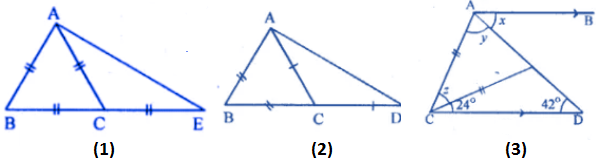Solution:

(a) in following figure

Given. ABC is an equilateral triangle BC = CE

To find. ∠ACE and ∠AEC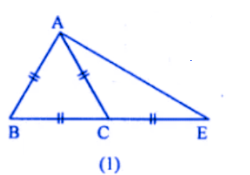As given that ABC is an equilateral triangle,

That is ∠BAC = ∠B = ∠ACB = 600 ……..(1)

(each angle of an equilateral triangle is 60o)

Now, ∠ACE = ∠BAC + CB

(Exterior angle is equal to sum of two interior opposite angles)

(∠ACE = 60o + 60o)

∠ACE = 1200

Then, in ∆ACE

Given, AC = CE … [because AC = BC = CE]

∠CAE = ∠AEC … (2)

We know that, in a triangle equal sides have equal angles opposite to them.

So, ∠CAE + ∠AEC + 120o = 80o

∠AEC + ∠AEC + 120o = 180o … [by equation (2) we get]

2∠AEC = 180o – 120o

2∠AEC = 60o

∠AEC = 60o/2

∠AEC = 30o

Therefore, ∠ACE = 120o and ∠AEC = 30o.

(b) In given figure,

Given, ∆ABD, AC meets BD in C. AB = BC, AC = CD.

Then, consider ∆ABC,

AB = BC … [given]

Therefore, ∠ACB = ∠BAC … (1)

(In a triangle, equal angles opposite to them)

In ∆ACD,

AC = CD … [given]

(In a triangle, equal sides have equal angles opposite to them)

From, adding (1) and (2), we get

Now, in ∆ACD

(In an triangle, exterior angle is equal to sum of two interior opposite angles)

(c) In given figure,

Given, AB parallel to CD, ∠ECD = 24o, ∠CDE = 42o

We have to find the value of x, y and z.

Consider, ∆CDE

Exterior, ∠CEA = 24o + 42o

(In a triangle exterior angle is equal to sum of two interior opposite angles)

∠CEA = 66o … (1)

Then, in ∆ACE

AC = CE … (given)

Therefore, ∠CAE = ∠CEA

(In a triangle equal side have equal angles opposite to them)

By equation (1),

Y = 66o … (2)

Also, y + z + ∠CEA = 180o

We know that, sum of all angles in a triangle is 180o

66o + z + 66o = 180o

z + 132o = 180o

z = 180o – 132o

z = 48o … (3)

Then it is given that, AB is parallel to CD,

∠x = ∠ADC … [alternate angles]

x = 42o … (4)

Therefore, from (2), (3) and (4) equation gives x = 42o, y = 66o and z = 48o.

6. In the given figure, AD, BE and CF arc altitudes of ∆ABC. If AD = BE = CF, prove that ABC is an equilateral triangle.

Given : in the figure given,

AD, BE and CF are altitudes of ∆ABC and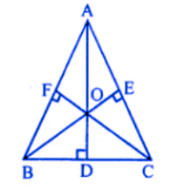To prove : ∆ABC is an equilateral triangle

Proof: in the right ∆BEC and ∆BFC

Hypotenuse BC = BC (Common)

Side BE = CF (Given)

∆BEC ≅ ∆BFC (RHS axiom)

∠C = ∠B

AB = AC (sides opposite to equal angles)

Similarly we can prove that ∆CFA ≅ ∆ADC

∠A = ∠C

AB = BC

From (i) and (ii)

AB = BC = AC

∆ABC is an equilateral triangle

7. In the given figure, D is mid-point of BC, DE and DF are perpendiculars to AB and AC respectively such that DE = DF. Prove that ABC is an isosceles triangle.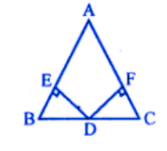Solution:

In triangle ABC

D is the midpoint of BC

DE perpendicular to AB

And DF perpendicular to AC

DE = DE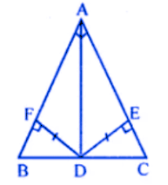To prove:

Triangle ABC is an isosceles triangle

Proof:

In the right angled triangle BED and CDF

Hypotenuse BD = DC (because D is a midpoint)

Side DF = DE (given)

∆BED ≅ ∆CDF (RHS axiom)

∠C = ∠B

AB = AC (sides opposite to equal angles)

∆ABC is an isosceles triangle

Exercise 10.4

1. In ∆PQR, ∠P = 70° and ∠R = 30°. Which side of this triangle is longest? Give reason for your answer.

Solution:

In ∆PQR, ∠P = 70o, ∠R = 30o

But ∠P + ∠Q + ∠R = 180o

100o + ∠Q = 180o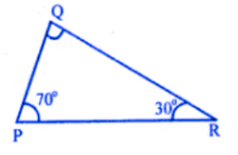∠Q = 180o – 100o = 180o

∠Q = 80o the greatest angle

Its opposite side PR is the longest side

(side opposite to greatest angle is longest)

2. Show that in a right angled triangle, the hypotenuse is the longest side.

Solution:

Given: in right angled ∆ABC, ∠B = 90o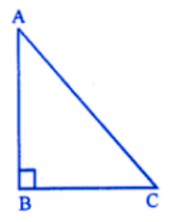To prove: AC is the longest side

Proof : in ∆ABC,

∠B = 90o

∠A and ∠C are acute angles

That is less then 90o

∠B is the greatest angle

Or ∠B> ∠C and ∠B> ∠A

AC > AB and AC > BC

Hence AC is the longest side

3. PQR is a right angle triangle at Q and PQ : QR = 3:2. Which is the least angle.

Solution: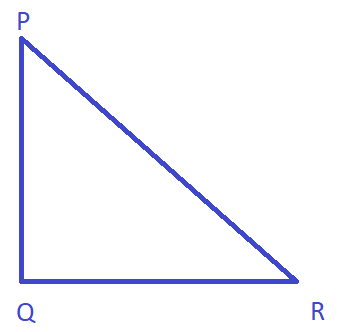Here, PQR is a right angle triangle at Q. Also given that

PQ : QR = 3:2

Let PQ = 3x, then, QR = 2x

It is clear that QR is the least side,

Then, we know that the least angle has least side

Opposite to it.

Hence ∠P is the least angle

4. In ∆ ABC, AB = 8 cm, BC = 5.6 cm and CA = 6.5 cm. Which is (i) the greatest angle ? (ii) the smallest angle ?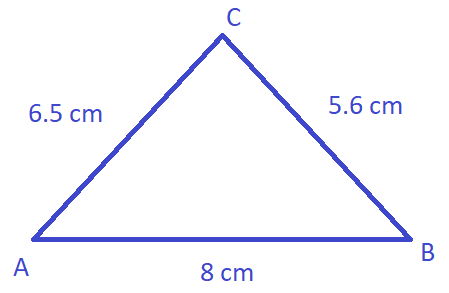Solution:

Given that AB = 8 cm, BC = 5.6 cm, CA = 6.5 cm.

Here AB is the greatest side

Then ∠C is the least angle

The greatest side has greatest angle opposite to it)

Also, BC Is the least side

Then ∠A is the least angle

(the least side has least angle opposite to it)

Chapter test

1. In triangles ABC and DEF, ∠A = ∠D, ∠B = ∠E and AB = EF. Will the two triangles be congruent? Give reasons for your answer.

Solution:

In ∆ABC and ∆DEF

∠A = ∠D

∠B = ∠E

AB = EF

In ∆ABC, two angles and included side is

Given but in ∆DEF, corresponding angles are

Equal but side is not included of there angle.

Triangles Cannot be congruent.

2. In the adjoining figure, ABCD is a square. P, Q and R are points on the sides AB, BC and CD respectively such that AP= BQ = CR and ∠PQR = 90°. Prove that (a) ∆PBQ ≅ ∆QCR (b) PQ = QR (c) ∠PRQ = 45°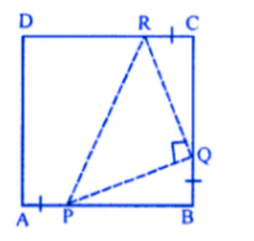Solution:

Given : in the given figure, ABCD is a square

P,Q and R are the Points on the sides AB,

BC and CD respectively such that

AP = BQ = CR, ∠PQR = 90o

To prove: (a) ∆PBQ = ∆QCR

(b) PQ = QR

(c) ∠PQR = 45o

Proof : AB = BC = CD (Sides of Square)

And AP = BQ = CR (Given)

Subtracting, we get

AB – AP = BC – BQ = CD – CR

(PB = QC = RD)

Now in ∆PBQ and ∠QCR

PB = QC (Proved)

BQ = CR (Given)

∠B = ∠C (Each 90o)

∆PBQ ≅ ∆QCR

PQ = QR

But ∠PQR = 90o

∠RPQ = ∠PQR

(Angles opposite to equal angles)

But ∠RPQ + ∠PRQ = 90o

∠RPQ = ∠PQR = 90o

∠RPQ = ∠PRQ = 90o/2 = 45o

3. In the given figure, OA ⊥ OD, OC X OB, OD = OA and OB = OC. Prove that AB = CD.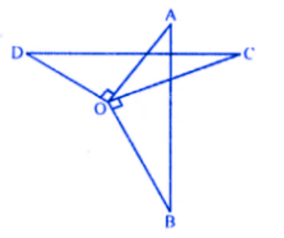Solution :

Given : in the figure OA ⊥ OD, OC ⊥ OB.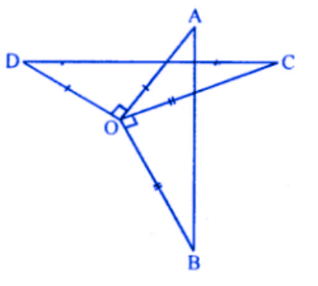To prove : AB = CD

Proof : ∠AOD = ∠COB (each 90o)

∠AOD + ∠AOC = ∠AOC + ∠COB

∠COD = ∠AOB

Now, in ∆AOB and ∆DOC

OA = OD (Given)

OB = OC (Given)

∠AOB = ∠COD (Proved)

∆AOB ≅ ∆DOC

AB = CD

4. In the given figure, PQ || BA and RS CA. If BP = RC, prove that: (i) ∆BSR ≅ ∆PQC (ii) BS = PQ (iii) RS = CQ.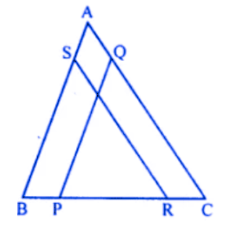Solution:

PQ ∥ BA, RS ∥ CA

BP = RC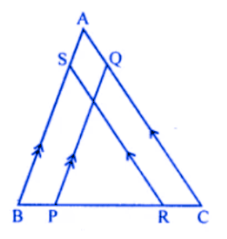To prove :

(i) ∆BSR ≅ ∆PQC

(ii) RS = CQ

Proof : BP = RC

BC – RC = BC – BP

BR = PC

Now, in ∆BSR and ∆PQC

∠B = ∠P (Corresponding angles)

∠R = ∠C (Corresponding angles)

BR = PC (Proved)

∆BSR ≅ ∆PQC

BS = PQ

RS = CQ